# US10578192B2 - Hypoid gear design method and hypoid gear - Google Patents

## Info

Publication number
US10578192B2
US10578192B2 US15/980,464 US201815980464A US10578192B2 US 10578192 B2 US10578192 B2 US 10578192B2 US 201815980464 A US201815980464 A US 201815980464A US 10578192 B2 US10578192 B2 US 10578192B2
Authority
US
United States
Prior art keywords
gear
γ
ψ
pitch
contact
Prior art date
Legal status (The legal status is an assumption and is not a legal conclusion. Google has not performed a legal analysis and makes no representation as to the accuracy of the status listed.)
Active
Application number
US15/980,464
Other versions
US20180259038A1 (en
Inventor
Takayuki Aoyama
Mizuho Inagaki
Kiyokazu Sunami
Sho Honda
Yoshikatsu Shibata
Hiromi Miyamura
Current Assignee (The listed assignees may be inaccurate. Google has not performed a legal analysis and makes no representation or warranty as to the accuracy of the list.)
Toyota Motor Corp
Toyota Central R&D Labs Inc
Original Assignee
Toyota Motor Corp
Toyota Central R&D Labs Inc
Priority date (The priority date is an assumption and is not a legal conclusion. Google has not performed a legal analysis and makes no representation as to the accuracy of the date listed.)
Filing date
Publication date
Priority to JP2008-187965 priority Critical
Priority to JP2008187965 priority
Priority to JP2008-280558 priority
Priority to JP2008280558 priority
Priority to JP2009111881 priority
Priority to JP2009-111881 priority
Priority to PCT/JP2009/063234 priority patent/WO2010008096A1/en
Priority to US201113054323A priority
Priority to US14/508,422 priority patent/US20150020626A1/en
Priority to US15/980,464 priority patent/US10578192B2/en
Application filed by Toyota Motor Corp, Toyota Central R&D Labs Inc filed Critical Toyota Motor Corp
Publication of US20180259038A1 publication Critical patent/US20180259038A1/en
Application granted granted Critical
Publication of US10578192B2 publication Critical patent/US10578192B2/en
Active legal-status Critical Current
Anticipated expiration legal-status Critical

• 238000004364 calculation methods Methods 0.000 description 16
• 239000000203 mixtures Substances 0.000 description 2
• 238000006243 chemical reaction Methods 0.000 description 1
• 238000004590 computer program Methods 0.000 description 1
• 238000009795 derivation Methods 0.000 description 1
• 238000004519 manufacturing process Methods 0.000 description 1
• 238000000034 methods Methods 0.000 description 1

## Images

•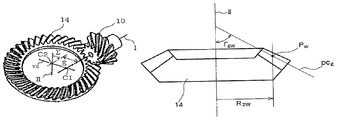•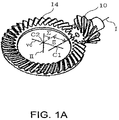•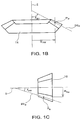•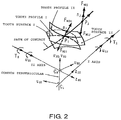•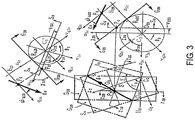•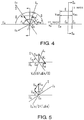•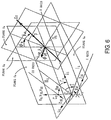•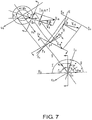•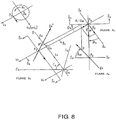•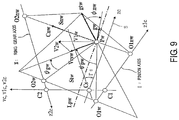•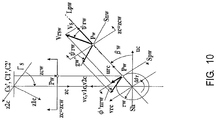•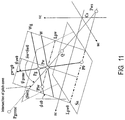•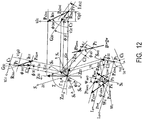•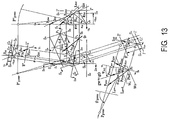•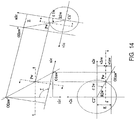•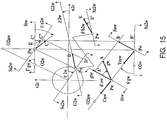•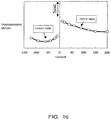•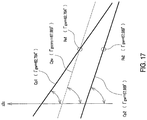•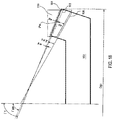•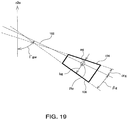•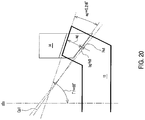•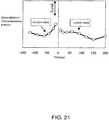••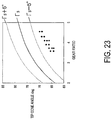•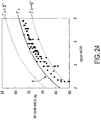•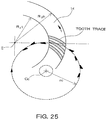•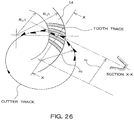## Classifications

• FMECHANICAL ENGINEERING; LIGHTING; HEATING; WEAPONS; BLASTING
• F16ENGINEERING ELEMENTS AND UNITS; GENERAL MEASURES FOR PRODUCING AND MAINTAINING EFFECTIVE FUNCTIONING OF MACHINES OR INSTALLATIONS; THERMAL INSULATION IN GENERAL
• F16HGEARING
• F16H1/00Toothed gearings for conveying rotary motion
• F16H1/02Toothed gearings for conveying rotary motion without gears having orbital motion
• F16H1/04Toothed gearings for conveying rotary motion without gears having orbital motion involving only two intermeshing members
• F16H1/12Toothed gearings for conveying rotary motion without gears having orbital motion involving only two intermeshing members with non-parallel axes
• F16H1/14Toothed gearings for conveying rotary motion without gears having orbital motion involving only two intermeshing members with non-parallel axes comprising conical gears only
• F16H1/145Toothed gearings for conveying rotary motion without gears having orbital motion involving only two intermeshing members with non-parallel axes comprising conical gears only with offset axes, e.g. hypoïd gearings
• FMECHANICAL ENGINEERING; LIGHTING; HEATING; WEAPONS; BLASTING
• F16ENGINEERING ELEMENTS AND UNITS; GENERAL MEASURES FOR PRODUCING AND MAINTAINING EFFECTIVE FUNCTIONING OF MACHINES OR INSTALLATIONS; THERMAL INSULATION IN GENERAL
• F16HGEARING
• F16H55/00Elements with teeth or friction surfaces for conveying motion; Worms; Pulleys; Sheaves
• F16H55/02Toothed members; Worms
• F16H55/08Profiling
• F16H55/0853Skewed-shaft arrangement of the toothed members
• FMECHANICAL ENGINEERING; LIGHTING; HEATING; WEAPONS; BLASTING
• F16ENGINEERING ELEMENTS AND UNITS; GENERAL MEASURES FOR PRODUCING AND MAINTAINING EFFECTIVE FUNCTIONING OF MACHINES OR INSTALLATIONS; THERMAL INSULATION IN GENERAL
• F16HGEARING
• F16H57/00General details of gearing
• F16H2057/0087Computer aided design [CAD] specially adapted for gearing features ; Analysis of gear systems
• YGENERAL TAGGING OF NEW TECHNOLOGICAL DEVELOPMENTS; GENERAL TAGGING OF CROSS-SECTIONAL TECHNOLOGIES SPANNING OVER SEVERAL SECTIONS OF THE IPC; TECHNICAL SUBJECTS COVERED BY FORMER USPC CROSS-REFERENCE ART COLLECTIONS [XRACs] AND DIGESTS
• Y10TECHNICAL SUBJECTS COVERED BY FORMER USPC
• Y10TTECHNICAL SUBJECTS COVERED BY FORMER US CLASSIFICATION
• Y10T29/00Metal working
• Y10T29/49Method of mechanical manufacture
• Y10T29/49462Gear making
• YGENERAL TAGGING OF NEW TECHNOLOGICAL DEVELOPMENTS; GENERAL TAGGING OF CROSS-SECTIONAL TECHNOLOGIES SPANNING OVER SEVERAL SECTIONS OF THE IPC; TECHNICAL SUBJECTS COVERED BY FORMER USPC CROSS-REFERENCE ART COLLECTIONS [XRACs] AND DIGESTS
• Y10TECHNICAL SUBJECTS COVERED BY FORMER USPC
• Y10TTECHNICAL SUBJECTS COVERED BY FORMER US CLASSIFICATION
• Y10T29/00Metal working
• Y10T29/49Method of mechanical manufacture
• Y10T29/49462Gear making
• Y10T29/49467Gear shaping
• YGENERAL TAGGING OF NEW TECHNOLOGICAL DEVELOPMENTS; GENERAL TAGGING OF CROSS-SECTIONAL TECHNOLOGIES SPANNING OVER SEVERAL SECTIONS OF THE IPC; TECHNICAL SUBJECTS COVERED BY FORMER USPC CROSS-REFERENCE ART COLLECTIONS [XRACs] AND DIGESTS
• Y10TECHNICAL SUBJECTS COVERED BY FORMER USPC
• Y10TTECHNICAL SUBJECTS COVERED BY FORMER US CLASSIFICATION
• Y10T29/00Metal working
• Y10T29/49Method of mechanical manufacture
• Y10T29/49462Gear making
• Y10T29/49467Gear shaping
• Y10T29/49476Gear tooth cutting
• YGENERAL TAGGING OF NEW TECHNOLOGICAL DEVELOPMENTS; GENERAL TAGGING OF CROSS-SECTIONAL TECHNOLOGIES SPANNING OVER SEVERAL SECTIONS OF THE IPC; TECHNICAL SUBJECTS COVERED BY FORMER USPC CROSS-REFERENCE ART COLLECTIONS [XRACs] AND DIGESTS
• Y10TECHNICAL SUBJECTS COVERED BY FORMER USPC
• Y10TTECHNICAL SUBJECTS COVERED BY FORMER US CLASSIFICATION
• Y10T74/00Machine element or mechanism
• Y10T74/19Gearing
• Y10T74/19642Directly cooperating gears
• Y10T74/1966Intersecting axes
• Y10T74/19665Bevel gear type
• YGENERAL TAGGING OF NEW TECHNOLOGICAL DEVELOPMENTS; GENERAL TAGGING OF CROSS-SECTIONAL TECHNOLOGIES SPANNING OVER SEVERAL SECTIONS OF THE IPC; TECHNICAL SUBJECTS COVERED BY FORMER USPC CROSS-REFERENCE ART COLLECTIONS [XRACs] AND DIGESTS
• Y10TECHNICAL SUBJECTS COVERED BY FORMER USPC
• Y10TTECHNICAL SUBJECTS COVERED BY FORMER US CLASSIFICATION
• Y10T74/00Machine element or mechanism
• Y10T74/19Gearing
• Y10T74/1987Rotary bodies

## Abstract

A degree of freedom of a hypoid gear is improved. An instantaneous axis in a relative rotation of a gear axis and a pinion axis, a line of centers, an intersection between the instantaneous axis and the line of centers, and an inclination angle of the instantaneous axis with respect to the rotation axis of the gear are calculated based on a shaft angle, an offset, and a gear ratio of a hypoid gear. Based on these variables, base coordinate systems are determined, and the specifications are calculated using these coordinate systems. For the spiral angles, pitch cone angles, and reference circle radii of the gear and pinion, one of the values for the gear and the pinion is set and a design reference point is calculated. Based on the design reference point and a contact normal of the gear, specifications are calculated. The pitch cone angle of the gear or the pinion can be freely selected.

## Description

The present application is a divisional application of U.S. application Ser. No. 14/508,422, filed on Oct. 7, 2014, which is a divisional application of U.S. application Ser. No. 13/054,323, filed Feb. 3, 2011, which is a National Stage Entry of PCT/JP2009/063234, filed Jul. 16, 2009, which claims priority to each of JP 2009-111881, filed May 1, 2009, JP 2008-280558, filed Oct. 30, 2008, and JP 2008-187965, filed Jul. 18, 2008. The disclosures of each of the above applications are hereby incorporated by reference in their entireties.

TECHNICAL FIELD

The present invention relates to a method of designing a hypoid gear.

BACKGROUND ART

A design method of a hypoid gear is described in Ernest Wildhaber, Basic Relationship of Hypoid Gears, American Machinist, USA, Feb. 14, 1946, p. 108-111 and in Ernest Wildhaber, Basic Relationship of Hypoid Gears II, American Machinist, USA, Feb. 28, 1946, p. 131-134. In these references, a system of eight equations is set and solved (for cone specifications that contact each other) by setting a spiral angle of a pinion and an equation of a radius of curvature of a tooth trace, in order to solve seven equations with nine variables which are obtained by setting, as design conditions, a shaft angle, an offset, a number of teeth, and a ring gear radius. Because of this, the cone specifications such as the pitch cone angle Γgw depend on the radius of curvature of the tooth trace, and cannot be arbitrarily determined.

In addition, in the theory of gears in the related art, a tooth trace is defined as “an intersection between a tooth surface and a pitch surface”. However, in the theory of the related art, there is no common geometric definition of a pitch surface for all kinds of gears. Therefore, there is no common definition of the tooth trace and of contact ratio of the tooth trace for various gears from cylindrical gears to hypoid gears. In particular, in gears other than the cylindrical gear and a bevel gear, the tooth trace is not clear.

In the related art, the contact ratio mf of tooth trace is defined by the following equation for all gears.
m f =F tan ψ0 /p
where, p represents the circular pitch, F represents an effective face width, and ψ0 represents a spiral angle.

Table 1 shows an example calculation of a hypoid gear according to the Gleason method. As shown in this example, in the Gleason design method, the tooth trace contact ratios are equal for a drive-side tooth surface and for a coast-side tooth surface. This can be expected because of the calculation of the spiral angle ψ0 as a virtual spiral bevel gear with ψ0=(ψpwgw)/2 (refer to FIG. 9).

The present inventors, on the other hand, proposed in Japanese Patent No. 3484879 a method for uniformly describing the tooth surface of a pair of gears. In other word, a method for describing a tooth surface has been shown which can uniformly be used in various situations from a pair of gears having parallel axes, which is the most widely used configuration, to a pair of gears whose axes do not intersect and are not parallel with each other (skew position).

There is a desire to determine the cone specifications independent from the radius of curvature of the tooth trace, and to increase the degree of freedom of the design.

In addition, in a hypoid gear, the contact ratio and the transmission error based on the calculation method of the related art are not necessarily correlated to each other. Of the contact ratios of the related art, the tooth trace contact ratio has the same value between the drive-side and the coast-side, and thus the theoretical basis is brought into question.

An advantage of the present invention is that a hypoid gear design method is provided which uses the uniform describing method of the tooth surface described in JP 3484879, and which has a high degree of freedom of design.

Another advantage of the present invention is that a hypoid gear design method is provided in which a design reference body of revolution (pitch surface) which can be applied to the hypoid gear, the tooth trace, and the tooth trace contact ratio are newly defined using the uniform describing method of the tooth surface described in JP 3484879, and the newly defined tooth trace contact ratio is set as a design index.

SUMMARY OF THE INVENTION

According to one aspect of the present invention, there is provided a design method of a hypoid gear wherein an instantaneous axis S which is an axis of a relative angular velocity of a first gear and a second gear, a line of centers vc which is common to rotation axes of the first gear and the second gear, an intersection Cs between the instantaneous axis S and the line of centers vc, and an inclination angle Γs of the instantaneous axis S with respect to the rotation axis of the second gear are calculated based on a shaft angle Σ, an offset E, and a gear ratio io of a hypoid gear, basic coordinate systems C1, C2, and Cs are determined from these variables, and specifications are calculated based on the coordinate systems. In particular, the specifications are calculated by setting a common point of contact of pitch cones of the first gear and the second gear as a design reference point Pw.

When an arbitrary point (design reference point) Pw is set in a static space, six cone specifications which are in contact at the point Pw are represented by coordinates (ucw, vcw, zcw) of the point Pw based on a plane (pitch plane) St defined by a peripheral velocity V1w and a peripheral velocity V2w at the point Pw and a relative velocity Vrsw. Here, the cone specifications refer to reference circle radii R1w and R2w of the first gear and the second gear, spiral angles ψpw and ψgw of the first gear and the second gear, and pitch cone angles γpw and Γgw of the first gear and the second gear. When three of these cone specifications are set, the point Pw is set, and thus the remaining three variables are also set. In other words, in various aspects of the present invention, the specifications of cones which contact each other are determined based merely on the position of the point Pw regardless of the radius of curvature of the tooth trace.

Therefore, it is possible to set a predetermined performance as a design target function, and select the cone specifications which satisfy the target function with a high degree of freedom. Examples of the design target function include, for example, a sliding speed of the tooth surface, strength of the tooth, and the contact ratio. The performance related to the design target function is calculated while the cone specification, for example the pitch cone angle Γgw, is changed, and the cone specification is changed and a suitable value is selected which satisfies the design request.

According to one aspect of the present invention, an contact ratio is employed as the design target function, and there is provided a method of designing a hypoid gear wherein a pitch cone angle Γgcone of one gear is set, an contact ratio is calculated, the pitch cone angle Γgcone is changed so that the contact ratio becomes a predetermined value, a pitch cone angle Γgw is determined, and specifications are calculated based on the determined pitch cone angle Γgw. As described above, the contact ratio calculated by the method of the related art does not have a theoretical basis. In this aspect of the present invention, a newly defined tooth trace and an contact ratio related to the tooth trace are calculated, to determine the pitch cone angle. The tooth surface around a point of contact is approximated by its tangential plane, and a path of contact is made coincident to an intersection of the surface of action (pitch generating line Lpw), and a tooth trace is defined as a curve on a pitch hyperboloid obtained by transforming the path of contact into a coordinate system which rotates with each gear. Based on the tooth trace of this new definition, the original contact ratio of the hypoid gear is calculated and the contact ratio can be used as an index for design. A characteristic of the present invention is in the definition of the pitch cone angle related to the newly defined tooth trace.

According to another aspect of the present invention, it is preferable that, in the hypoid gear design method, the tooth trace contact ratio is assumed to be 2.0 or more in order to achieve constant engagement of two gears with two or more teeth.

When the pitch cone angle Γgw is set to an inclination angle Γs of an instantaneous axis, the contact ratios of the drive-side and the coast-side can be set approximately equal to each other. Therefore, it is preferable for the pitch cone angle to be set near the inclination angle of the instantaneous axis. In addition, it is also preferable to increase one of the contact ratios of the drive-side or coast-side as required. In this process, first, the pitch cone angle is set at the inclination angle of the instantaneous axis and the contact ratio is calculated, and a suitable value is selected by changing the pitch cone angle while observing the contact ratio. It is preferable that a width of the change of the pitch cone angle be in a range of ±5° with respect to the inclination angle Γs of the instantaneous axis. This is because if the change is out of this range, the contact ratio of one of the drive-side and the coast-side will be significantly reduced.

More specifically, according to one aspect of the present invention, a hypoid gear is designed according to the following steps:

(a) setting a shaft angle Σ, an offset E, and a gear ratio i0 of a hypoid gear;

(b) calculating, based on the shaft angle Σ, the offset E, and the gear ratio i0, an instantaneous axis S which is an axis of a relative angular velocity of a first gear and a second gear, a line of centers vc with respect to rotation axes of the first gear and the second gear, an intersection Cs between the instantaneous axis S and the line of centers vc, and an inclination angle Γs of the instantaneous axis S with respect to the rotation axis of the second gear, and determining coordinate systems C1, C2, and Cs for calculation of specifications;

(c) setting three variables including one of a reference circle radius R1w of the first gear and a reference circle radius R2w of the second gear, one of a spiral angle ψpw of the first gear and a spiral angle ψgw of the second gear, and one of a pitch cone angle γpw of the first gear and a pitch cone angle Γgw of the second gear;

(d) calculating the design reference point Pw, which is a common point of contact of pitch cones of the first gear and the second gear, and the three other variables which are not set in the step (c), based on the three variables which are set in the step (c);

(e) setting a contact normal gwD of a drive-side tooth surface of the second gear;

(f) setting a contact normal gwC of a coast-side tooth surface of the second gear; and

(g) calculating specifications of the hypoid gear based on the design reference point Pw, the three variables which are set in the step (c), the contact normal gwD of the drive-side tooth surface of the second gear, and the contact normal gwC of the coast-side tooth surface of the second gear.

According to another aspect of the present invention, a hypoid gear is designed according to the following steps:

(a) setting a shaft angle Σ, an offset E, and a gear ratio i0 of a hypoid gear;

(b) calculating, based on the shaft angle Σ, the offset E, and the gear ratio i0, an instantaneous axis S which is an axis of a relative angular velocity of a first gear and a second gear, a line of centers vc with respect to rotation axes of the first gear and the second gear, an intersection Cs between the instantaneous axis S and the line of centers vc, and an inclination angle Γs of the instantaneous axis S with respect to the rotation axis of the second gear, and determining coordinate systems C1, C2, and Cs for calculation of specifications;

(c) setting three variables including one of a reference circle radius R1W of the first gear and a reference circle radius R2w of the second gear, one of a spiral angle ψpw of the first gear and a spiral angle ψgw of the second gear, and one of a pitch cone angle γpw of the first gear and a pitch cone angle Γgw of the second gear;

(d) calculating the design reference point Pw, which is a common point of contact of pitch cones of the first gear and the second gear, and the three other variables which are not set in the step (c), based on the three variable which are set in the step (c);

(e) calculating a pitch generating line Lpw which passes through the design reference point Pw and which is parallel to the instantaneous axis S;

(f) setting an internal circle radius R2t and an external circle radius R2h of the second gear;

(g) setting a contact normal gwD of a drive-side tooth surface of the second gear;

(h) calculating an intersection P0D between a reference plane SH which is a plane orthogonal to the line of centers vc and passing through the intersection Cs and the contact normal gwD and a radius R20D of the intersection POD around a gear axis;

(i) calculating an inclination angle φs0D of a surface of action SwD which is a plane defined by the pitch generating line Lpw and the contact normal gwD with respect to the line of centers vc, an inclination angle ψsw0D of the contact normal gwD on the surface of action SwD with respect to the instantaneous axis S, and one pitch PgwD on the contact normal gwD;

(j) setting a provisional second gear pitch cone angle Γgcone, and calculating an contact ratio mfconeD of the drive-side tooth surface based on the internal circle radius R2t and the external circle radius R2h;

(k) setting a contact normal gwC of a coast-side tooth surface of the second gear;

(l) calculating an intersection P0C between the reference plane SH which is a plane orthogonal to the line of centers vc and passing through the intersection Cs and the contact normal gwC and a radius R20c of the intersection P0C around the gear axis;

(m) calculating an inclination angle ϕs0C of a surface of action SwC which is a plane defined by the pitch generating line Lpw and the contact normal gwC with respect to the line of centers vc, an inclination angle ψsw0C of the contact normal gwC on the surface of action SwC with respect to the instantaneous axis S, and one pitch PgwC on the contact normal gwC;

(n) setting a provisional second gear pitch cone angle Γgcone, and calculating an contact ratio mfconeC of the coast-side tooth surface based on the internal circle radius R2t and the external circle radius R2h;

(o) comparing the contact ratio mfconeD of the drive-side tooth surface and the contact ratio mfconeC of the coast-side tooth surface, and determining whether or not these contact ratios are predetermined values;

(p) when the contact ratios of the drive-side and the coast-side are the predetermined values, replacing the provisional second gear pitch cone angle Γgcone with the second gear pitch cone angle Γgw obtained in the step (c) or in the step (d);

(q) when the contact ratios of the drive-side and the coast-side are not the predetermined values, changing the provisional second gear pitch cone angle Γgcone and re-executing from step (g);

(r) re-determining the design reference point Pw, the other one of the reference circle radius R1w of the first gear and the reference circle radius R2w of the second gear which is not set in the step (c), the other one of the spiral angle ψpw of the first gear and the spiral angle ψgw of the second gear which is not set in the step (c), and the first gear pitch cone angle γpw based on the one of the reference circle radius R1w of the first gear and the reference circle radius R2w of the second gear which is set in the step (c), the one of the spiral angle ψpw of the first gear and the spiral angle ψgw of the second gear which is set in the step (c), and the second gear pitch cone angle Γgw which is replaced in the step (p), and

(s) calculating specifications of the hypoid gear based on the specifications which are set in the step (c), the specifications which are re-determined in the step (r), the contact normal gwD of the drive-side tooth surface of the second gear, and the contact normal gwC of the coast-side tooth surface of the second gear.

According to another aspect of the present invention, a hypoid gear is designed according to the following steps:

(a) setting a shaft angle Σ, an offset E, and a gear ratio i0 of a hypoid gear;

(b) calculating, based on the shaft angle Σ, the offset E, and the gear ratio i0, an instantaneous axis S which is an axis of a relative angular velocity of a first gear and a second gear, a line of centers vc with respect to rotation axes of the first gear and the second gear, an intersection Cs between the instantaneous axis S and the line of centers vc, and an inclination angle Γs of the instantaneous axis S with respect to the rotation axis of the second gear, to determine coordinate systems C1, C2, and Cs for calculation of specifications;

(c) setting three variables including one of a reference circle radius R1w of the first gear and a reference circle radius R2w of the second gear, one of a spiral angle ψpw of the first gear and a spiral angle ψgw of the second gear, and one of a pitch cone angle γpw of the first gear and a pitch cone angle Γgw of the second gear;

(d) calculating the design reference point Pw, which is a common point of contact of pitch cones of the first gear and the second gear, and the three other variables which are not set in the step (c), based on the three variables which are set in the step (c);

(e) calculating a pitch generating line Lpw which passes through the design reference point Pw and which is parallel to the instantaneous axis S;

(f) setting an internal circle radius R2t and an external circle radius R2h of the second gear;

(g) setting a contact normal gwD of a drive-side tooth surface of the second gear;

(h) calculating an intersection P0D between a reference plane SH which is a plane orthogonal to the line of centers vc and passing through the intersection Cs and the contact normal gwD and a radius R20D of the intersection POD around a gear axis;

(i) calculating an inclination angle φs0D Of a surface of action SwD which is a plane defined by the pitch generating line Lpw and the contact normal gwD with respect to the line of centers vc, an inclination angle ψsw0D of the contact normal gwD on the surface of action SwD with respect to the instantaneous axis S, and one pitch PgWD on the contact normal gwD;

(j) setting a provisional second gear pitch cone angle Γgcone, and calculating an contact ratio mfconeD of the drive-side tooth surface based on the internal circle radius R2t and the external circle radius R2h;

(k) setting a contact normal gwC of a coast-side tooth surface of the second gear;

(l) calculating an intersection P0C between the reference plane SH which is a plane orthogonal to the line of centers vc and passing through the intersection Cs and the contact normal gwC and a radius R20c of the intersection P0C around the gear axis;

(m) calculating an inclination angle φs0C of a surface of action SwC which is a plane defined by the pitch generating line Lpw and the contact normal gwC with respect to the line of centers vc, an inclination angle ψsw0C of the contact normal gwC on the surface of action SwC with respect to the instantaneous axis S, and one pitch PgwC on the contact normal gwC;

(n) setting a provisional second gear pitch cone angle Γgcone, and calculating an contact ratio mfconeC of the coast-side tooth surface based on the internal circle radius R2t and the external circle radius R2h;

(o) comparing the contact ratio mfconeD of the drive-side tooth surface and the contact ratio mfconeC of the coast-side tooth surface, and determining whether or not these contact ratios are predetermined values;

(p) changing, when the contact ratios of the drive-side and the coast-side are not the predetermined values, the provisional second gear pitch cone angle Γgcone and re-executing from step (g);

(q) defining, when the contact ratios of the drive-side and the coast-side are the predetermined values, a virtual cone having the provisional second gear pitch cone angle Γgcone as a cone angle;

(r) calculating a provisional pitch cone angle γpcone of the virtual cone of the first gear based on the determined pitch cone angle Γgcone; and

(s) calculating specifications of the hypoid gear based on the design reference point Pw, the reference circle radius R1w of the first gear and the reference circle radius R2w of the second gear which are set in the step (c) and the step (d), the spiral angle ψpw of the first gear and the spiral angle ψgw of the second gear which are set in the step (c) and the step (d), the cone angle Γgcone of the virtual cone and the cone angle γpcone of the virtual cone which are defined in the step (q) and the step (r), the contact normal gwD of the drive-side tooth surface of the second gear, and the contact normal gwC of the coast-side tooth surface of the second gear.

According to another aspect of the present invention, in a method of designing a hypoid gear, a pitch cone angle of one gear is set equal to an inclination angle of an instantaneous axis, and the specifications are calculated. When the pitch cone angle is set equal to the inclination angle of the instantaneous axis, the contact ratios of the drive-side tooth surface and the coast-side tooth surface become almost equal to each other. Therefore, a method is provided in which the pitch cone angle is set to the inclination angle of the instantaneous axis in a simple method, that is, without reviewing the contact ratios in detail.

More specifically, according to another aspect of the present invention, the hypoid gear is designed according to the following steps:

(a) setting a shaft angle Σ, an offset E, and a gear ratio i0 of a hypoid gear;

(b) calculating, based on the shaft angle Σ, the offset E, and the gear ratio i0, an instantaneous axis S which is an axis of a relative angular velocity of a first gear and a second gear, a line of centers vc with respect to rotational axes of the first gear and the second gear, an intersection Cs between the instantaneous axis S and the line of centers vc, and an inclination angle Γs of the instantaneous axis with respect to the rotational axis of the second gear;

(c) determining the inclination angle Γs of the instantaneous axis as a second gear pitch cone angle Γgw; and

(d) calculating specifications of the hypoid gear based on the determined second gear pitch cone angle Γgw.

According to another aspect of the present invention, in a method of designing a hypoid gear, a design reference point Pw is not set as a point of contact between the pitch cones of the first gear and second gear, but is determined based on one of reference circle radii R1w and R2w of the first gear and the second gear, a spiral angle ψrw, and a phase angle βw of the design reference point, and the specifications are calculated.

More specifically, according to another aspect of the present invention, a hypoid gear is designed according to the following steps:

(a) setting a shaft angle Σ, an offset E, and a gear ratio i0 of a hypoid gear;

(b) calculating, based on the shaft angle Σ, the offset E, and the gear ratio i0, an instantaneous axis S which is an axis of a relative angular velocity of a first gear and a second gear, a line of centers vc with respect to rotation axes of the first gear and the second gear, an intersection Cs between the instantaneous axis S and the line of centers vc, and an inclination angle Γs of the instantaneous axis S with respect to the rotation axis of the second gear, to determine coordinate systems C1, C2, and Cs for calculation of specifications;

(c) setting one of a reference circle radius R1w of the first gear and a reference circle radius R2w of the second gear, a spiral angle ψrw, and a phase angle βw of a design reference point Pw, to determine the design reference point;

(d) calculating the design reference point Pw and a reference circle radius which is not set in the step (c) from a condition where the first gear and the second gear share the design reference point Pw, based on the three variables which are set in the step (c);

(e) setting one of a reference cone angle γpw of the first gear and a reference cone angle Γgw of the second gear;

(f) calculating a reference cone angle which is not set in the step (e), based on the shaft angle Σ and the reference cone angle which is set in the step (e);

(g) setting a contact normal gwD of a drive-side tooth surface of the second gear;

(h) setting a contact normal gwC of a coast-side tooth surface of the second gear; and

(i) calculating specifications of the hypoid gear based on the design reference point Pw, the reference circle radii R1w and R2w, and the spiral angle ψrw, which are set in the step (c) and the step (d), the reference cone angles γpw and Γgw which are set in the step (e) and the step (f), and the contact normals gwC and gwD which are set in the step (g) and the step (h).

The designing steps of these two aspects of the present invention can be executed by a computer by describing the steps with a predetermined computer program. A unit which receives the gear specifications and variables is connected to the computer and a unit which provides a design result or a calculation result at an intermediate stage is also connected to the computer.

BRIEF DESCRIPTION OF THE DRAWINGS

FIG. 1A is a diagram showing external appearance of a hypoid gear.

FIG. 1B is a diagram showing a cross sectional shape of a gear.

FIG. 1C is a diagram showing a cross sectional shape of a pinion.

FIG. 2 is a diagram schematically showing appearance of coordinate axes in each coordinate system, a tooth surface of a gear, a tooth profile, and a path of contact.

FIG. 3 is a diagram showing a design reference point P0 and a path of contact g0 for explaining a variable determining method, with coordinate systems C2′, Oq2, C1′, and Oq1.

FIG. 4 is a diagram for explaining a relationship between gear axes I and II and an instantaneous axis S.

FIG. 5 is a diagram showing a relative velocity Vs at a point Cs.

FIG. 6 is a diagram showing, along with planes SH, Ss, Sp, and Sn, a reference point P0, a relative velocity Vrs0, and a path of contact g0.

FIG. 7 is a diagram showing a relationship between a relative velocity Vrs and a path of contact g0 at a point P.

FIG. 8 is a diagram showing a relative velocity Vrs0 and a path of contact g0 at a reference point P0, with a coordinate system Cs.

FIG. 9 is a diagram showing coordinate systems C1, C2, and Cs of a hypoid gear and a pitch generating line Lpw.

FIG. 10 is a diagram showing a tangential cylinder of a relative velocity Vrsw.

FIG. 11 is a diagram showing a relationship between a pitch generating line Lpw, a path of contact gw, and a surface of action Sw at a design reference point Pw.

FIG. 12 is a diagram showing a surface of action using coordinate systems Cs, C1, and C2 in the cases of a cylindrical gear and a crossed helical gear.

FIG. 13 is a diagram showing a surface of action using coordinate systems Cs, C1, and C2 in the cases of a bevel gear and a hypoid gear.

FIG. 14 is a diagram showing a relationship between a contact point Pw and points O1nw and O2nw.

FIG. 15 is a diagram showing a contact point Pw and a path of contact gw in planes Stw, Snw, and G2w.

FIG. 16 is a diagram showing a transmission error of a hypoid gear manufactured as prototype that uses current design method.

FIG. 17 is an explanatory diagram of a virtual pitch cone.

FIG. 18 is a diagram showing a definition of a ring gear shape.

FIG. 19 is a diagram showing a definition of a ring gear shape.

FIG. 20 is a diagram showing a state in which an addendum is extended and a tip cone angle is changed.

FIG. 21 is a diagram showing a transmission error of a hypoid gear manufactured as prototype using a design method of a preferred embodiment of the present invention.

FIG. 22 is a schematic structural diagram of a system which aids a design method for a hypoid gear.

FIG. 23 is a diagram showing a relationship between a gear ratio and a tip cone angle of a uniform tooth depth hypoid gear designed using a current method.

FIG. 24 is a diagram showing a relationship between a gear ratio and a tip cone angle of a tapered tooth depth hypoid gear designed using a current method.

FIG. 25 is a diagram showing a relationship between a tooth trace curve and a cutter radius of a uniform-depth tooth.

FIG. 26 is a diagram showing a relationship between a tooth trace curve and a cutter radius of a tapered-depth tooth.

DESCRIPTION OF THE PREFERRED EMBODIMENT

A preferred embodiment of the present invention will now be described with reference to the drawings.

1. Coordinate System of Hypoid Gear

1.1 Coordinate Systems C1, C2, Cq1, and Cq2

In the following description, a small diameter gear in a pair of hypoid gears is referred to as a pinion, and a large diameter gear is referred to as a ring gear. In addition, in the following, the descriptions may be based on the tooth surface, tooth trace, etc. of the ring gear, but because the pinion and the ring gear are basically equivalent, the description may similarly be based on the pinion. FIG. 1A is a perspective view showing external appearance of a hypoid gear. The hypoid gear is a pair of gears in which a rotational axis (pinion axis) I of the pinion 10 and the rotational axis (gear axis) II of the ring gear 14 are not parallel and do not intersect. A line of centers vc of the pinion axis and the gear axis exists, and a distance (offset) between the two axes on the line of centers vc is set as E, an angle (shaft angle) between the pinion axis and the gear axis projected onto a plane orthogonal to the line of centers vc is set as E, and a gear ratio is set as i0. FIG. 1B is a cross sectional diagram at a plane including the axis II of the ring gear 14. An angle between a pitch cone element pcg passing at a design reference point Pw, to be described later, and the axis II is shown as a pitch cone angle Γgw. A distance between the design reference point Pw and the axis II is shown as a reference circle radius R2w. FIG. 1C is a cross sectional diagram at a plane including the axis I of the pinion 10. An angle between a pitch cone element pcp passing at the design reference point Pw and the axis I is shown as a pitch cone angle γpw. In addition, a distance between the design reference point Pw and the axis I is shown as a reference circle radius R1w.

FIG. 2 shows coordinate systems C1 and C2. A direction of the line of centers vc is set to a direction in which the direction of an outer product ω2i×ω1i of angular velocities ω1i and ω2i of the pinion gear axis I and the ring gear axis II is positive. The intersection points of the pinion and ring gear axes I, II and the line of centers vc are designated by C1 and C2 and a situation where C2 is above C1 with respect to the line of centers vc will be considered in the following. A case where C2 is below C1 would be very similar. A distance between C1 and C2 is the offset E. A coordinate system C2 of a ring gear 14 is defined in the following manner. The origin of the coordinate system C2 (u2c, v2c, z2c) is set at C2, a z2c axis of the coordinate system C2 is set to extend in the ω20 direction on the ring gear axis II, a v2c axis of the coordinate system C2 is set in the same direction as that of the line of centers vc, and a u2c axis of the coordinate system C2 is set to be normal to both the axes to form a right-handed coordinate system. A coordinate system C1 (u1c, v1c, z1c) can be defined in a very similar manner for the pinion 10.

FIG. 3 shows a relationship between the coordinate systems C1, C2, Cq1, and Cq2 in gears I and II. The coordinate systems C2 and Cq2 of the gear II are defined in the following manner. The origin of the coordinate system C2 (u2c, v2c, z2c) is set at C2, a z2c axis of the coordinate system C2 is set to extend in the ω20 direction on the ring gear axis II, a v2c axis of the coordinate system C2 is set in the same direction as that of the line of centers vc, and a u2c axis of the coordinate system C2 is set to be normal to both the axes to form a right-handed coordinate system. The coordinate system Cq2 (q2c, vq2c, z2c) has the origin C2 and the z2c axis in common, and is a coordinate system formed by the rotation of the coordinate system C2 around the z2c axis as a rotational axis by χ20 (the direction shown in the figure is positive) such that the plane v2c=0 is parallel to the plane of action G20. The u2c axis becomes a q2c axis, and the v2c axis becomes a vq2c axis.

The plane of action G20 is expressed by vq2c=−Rb2 using the coordinate system Cq2. In the coordinate system C2, the inclination angle of the plane of action G20 to the plane v2c=0 is the angle χ20, and the plane of action G20 is a plane tangent to the base cylinder (radius Rb20).

The relationships between the coordinate systems C2 and Cq2 become as follows because the z2c axis is common.
u 2c =q 2c cos χ20 −v q2c sin χ20
v 2c =q 2c sin χ20 +v q2c cos χ20

Because the plane of action G20 meets vq2c=−Rb20, the following expressions (1), are satisfied if the plane of action G20 is expressed by the radius Rb20 of the base cylinder.
u 2c =q 2c cos χ20 +R b20 sin χ20
v 2c =q 2c sin χ20 −R b20 cos χ20
z 2c =z 2c  (1)

If the line of centers g0 is defined to be on the plane of action G20 and also defined such that the line of centers g0 is directed in the direction in which the q2c axis component is positive, an inclination angle of the line of centers g0 from the q2c axis can be expressed by ψb20 (the direction shown in the figure is positive). Accordingly, the inclination angle of the line of centers g0 in the coordinate system C2 is defined to be expressed in the form of g0 20, ψb20) with the inclination angle φ20 (the complementary angle of the χ20) of the plane of action G20 with respect to the line of centers vc, and ψb2.

As for the gear I, coordinate systems C1 (u1c, v1c, z1c) and Cq1 (q1C, vq1c, z1c), a plane of action G10, a radius Rb1 of the base cylinder, and the inclination angle g0 10, ψb10) of the line of centers g0 can be similarly defined. Because the systems share a common z1c axis, the relationship between the coordinate systems C1 and Cq1 can also be expressed by the following expressions (2).
u 1c =q 1c cos χ10 +R b10 sin χ10
v 1c =q 1c sin χ10 −R b10 cos χ10
z 1c =z 1c  (2)

The relationship between the coordinate systems C1 and C2 is expressed by the following expressions (3).
u 1c =−u 2c cos Σ−z 2c sin Σ
v 1c =v 2c +E
z 1c =u 2c sin Σ−z 2c cos Σ  (3)
1.2 Instantaneous Axis (Relative Rotational Axis) S

FIG. 4 shows a relationship between an instantaneous axis and a coordinate system CS. If the orthogonal projections of the two axes I (ω10) and II (ω20) to the plane SH are designated by Is 10″) and IIs 20″), respectively, and an angle of Is with respect to IIS when the plane SH is viewed from the positive direction of the line of centers vc to the negative direction thereof is designated by Ω, Is is in a zone of 0≤Ω≤π (the positive direction of the angle Ω is the counterclockwise direction) with respect to IIS in accordance with the definition of ω20×ω10. If an angle of the instantaneous axis S (ωr) to the IIs on the plane SH is designated by ΩS (the positive direction of the angle ΩS is the counterclockwise direction), the components of ω10″ and ω20″ that are orthogonal to the instantaneous axis on the plane SH must be equal to each other in accordance with the definition of the instantaneous axis (ωr10−ω20). Consequently, Ωs satisfies the following expressions (4):
sin Ωs/sin(Ωs−Ω)=ω1020; or
sin Γs/sin(Σ−Γs)=ω1020  (4)
wherein Σ=π−Ω (shaft angle) and Γs=π−Ωs. The positive directions are shown in the figure. In other words, the angle Γs is an inclination of the instantaneous axis S with respect to the ring gear axis IIs on the plane SH, and the angle Γs will hereinafter be referred to as an inclination angle of the instantaneous axis.

The location of Cs on the line of centers vc can be obtained as follows. FIG. 5 shows a relative velocity Vs (vector) of the point Cs. In accordance with the aforesaid supposition, C1 is located under the position of C2 with respect to the line of centers vc and ω10≥ω20. Consequently, Cs is located under C2. If the peripheral velocities of the gears I, II at the point Cs are designated by Vs1 and Vs2 (both being vectors), respectively, because the relative velocity Vs (=Vs1−Vs2) exists on the instantaneous axis S, the components of Vs1 and Vs2 (existing on the plane SH) orthogonal to the instantaneous axis must always be equal to each other. Consequently, the relative velocity Vs (=Vs1−Vs2) at the point Cs would have the shapes as shown in the same figure on the plane SH according to the location (Γs) of the instantaneous axis S, and the distance C2Cs between C2 and Cs can be obtained by the following expression (5). That is,
C 2 C s =E tan Γs/{tan(Σ−Γs)+tan Γs}  (5).

The expression is effective within a range of 0≤Γs≤π, and the location of Cs changes together with Γs, and the location of the point Cs is located above C1 in the case of 0≤Γs≤π/2, and the location of the point Cs is located under C1 in the case of π/2≤Γs≤π.

1.3 Coordinate System Cs

Because the instantaneous axis S can be determined in a static space in accordance with the aforesaid expressions (4) and (5), the coordinate system Cs is defined as shown in FIG. 4. The coordinate system Cs(uc, vc, zc) is composed of Cs as its origin, the directed line of centers vc as its vc axis, the instantaneous axis S as its zc axis (the positive direction thereof is the direction of ωr), and its uc axis taken to be normal to both the axes as a right-handed coordinate system. Because it is assumed that a pair of gears being objects transmit a motion of a constant ratio of angular velocity, the coordinate system Cs becomes a coordinate system fixed in the static space, and the coordinate system Cs is a basic coordinate system in the case of treating a pair of gears performing the transmission of the motion of constant ratio of angular velocity together with the previously defined coordinate systems C1 and C2.

1.4 Relationship Among Coordinate Systems C1, C2, and Cs

If the points C1 and C2 are expressed to be C1 (0, vcs1, 0) and C2 (0, vcs2, 0) by the use of the coordinate system Cs, vcs1 and vcs2 are expressed by the following expressions (6).

$V cs ⁢ ⁢ 2 = C s ⁢ C 2 = E ⁢ ⁢ tan ⁢ ⁢ Γ s ⁢ / ⁢ { tan ⁡ ( Σ - Γ s ) + tan ⁢ ⁢ Γ s } ⁢ ⁢ V cs ⁢ ⁢ 1 = ⁢ C s ⁢ C 1 - V cs ⁢ ⁢ 2 - E = ⁢ - E ⁢ ⁢ tan ⁡ ( Σ - Γ s ) ⁢ / ⁢ { tan ⁡ ( Σ - Γ s ) + tan ⁢ ⁢ Γ s } ( 6 )$

If it is noted that C2 is always located above Cs with respect to the vc axis, the relationships among the coordinate system Cs and the coordinate systems C1 and C2 can be expressed as the following expressions (7) and (8) with the use of vcs1, vcs2, Σ, and Γs.
u 1c =u c cos(Σ−Γs)+z c sin(Σ−Γs)
v 1c =v c −v cs1
z 1c =−u c sin(Σ−Γs)+z c cos(Σ−Γs)  (7)
u 2c =−u c cos Γs +z c sin Γs
v 2c =v c −v cs2
z 2c =−u c sin Γs −z c cos Γs  (8)

The relationships among the coordinate system Cs and the coordinate systems C1 and C2 are conceptually shown in FIG. 6.

2. Definition of Path of Contact g0 by Coordinate System Cs

2.1 Relationship Between Relative Velocity and Path of Contact g0

FIG. 7 shows a relationship between the set path of contact g0 and a relative velocity Vrs (vector) at an arbitrary point P on g0. Incidentally, a prime sign (′) and a double-prime sign (″) in the figure indicate orthogonal projections of a point and a vector on the target plane. If the position vector of the P from an arbitrary point on the instantaneous axis S is designated by r when a tooth surface contacts at the arbitrary point P on the path of contact g0, the relative velocity Vrs at the point P can be expressed by the following expression (9).
v rsr ×r+V s  (9)
where
ωr10−ω20
ωr20 sin Σ/sin(Σ−Γs)=ω10 sin Σ/sin Γs
V s10×[C 1 C s]−ω20×[C 2 C s]
V s20 E sin Γs10 E sin(Σ−Γs)

Here, [C1Cs] indicates a vector having C1 as its starting point and Cs as its end point, and [C2Cs] indicates a vector having C2 as its starting point and Cs as its end point.

The relative velocity Vrs exists on a tangential plane of the surface of a cylinder having the instantaneous axis S as an axis, and an inclination angle ψ relative to Vs on the tangential plane can be expressed by the following expression (10).
cos ψ=|V s |/|V rs|  (10)

Because the path of contact g0 is also the line of centers of a tooth surface at the point of contact, g0 is orthogonal to the relative velocity Vrs at the point P. That is,
V rs ·g 0=0

Consequently, g0 is a directed straight line on a plane N normal to Vrs at the point P. If the line of intersection of the plane N and the plane SH is designated by Hn, Hn is in general a straight line intersecting with the instantaneous axis S, with g0 necessarily passing through the Hn if an infinite intersection point is included. If the intersection point of g0 with the plane SH is designated by P0, then P0 is located on the line of intersection Hn, and g0 and P0 become as follows according to the kinds of pairs of gears.

(1) Case of Cylindrical Gears or Bevel Gears (Σ=0, π or E=0)

Because Vs=0, Vrs simply means a peripheral velocity around the instantaneous axis S. Consequently, the plane N includes the S axis. Hence, Hn coincides with S, and the path of contact g0 always passes through the instantaneous axis S. That is, the point P0 is located on the instantaneous axis S. Consequently, for these pairs of gears, the path of contact g0 is an arbitrary directed straight line passing at the arbitrary point P0 on the instantaneous axis.

(2) Case of Gear Other than that Described Above (Σ≠0, π or E≠0)

In the case of a hypoid gear, a crossed helical gear or a worm gear, if the point of contact P is selected at a certain position, the relative velocity Vrs, the plane N, and the straight line Hn, all peculiar to the point P, are determined. The path of contact g0 is a straight line passing at the arbitrary point P0 on Hn, and does not, in general, pass through the instantaneous axis S. Because the point P is arbitrary, g0 is also an arbitrary directed straight line passing at the point P0 on a plane normal to the relative velocity Vrs0 at the intersection point P0 with the plane SH. That is, the aforesaid expression (9) can be expressed as follows.
V rs =V rs0r×[P 0 Pg 0

Here, [P0P] indicates a vector having P0 as its starting point and the P as its end point. Consequently, if Vrs0·g0=0, Vrs·g0=0, and the arbitrary point P on g0 is a point of contact.

2.2 Selection of Reference Point

Among pairs of gears having two axes with known positional relationship and the angular velocities, pairs of gears with an identical path of contact g0 have an identical tooth profile corresponding to g0, with the only difference between them being which part of the tooth profile issued effectively. Consequently, in design of a pair of gears, the position at which the path of contact g0 is disposed in a static space determined by the two axes is important. Further, because a design reference point is only a point for defining the path of contact g0 in the static space, the position at which the design reference point is selected on the path of contact g0 does not cause any essential difference. When an arbitrary path of contact g0 is set, the g0 necessarily intersects with a plane SH including the case where the intersection point is located at an infinite point. Thus, the path of contact g0 is determined with the point P0 on the plane SH (on an instantaneous axis in the case of cylindrical gears and bevel gears) as the reference point.

FIG. 8 shows the reference point P0 and the path of contact g0 by the use of the coordinate system Cs. When the reference point expressed by means of the coordinate system Cs is designated by P0 (uc0, vc0, zc0), each coordinate value can be expressed as follows.
u c0 =O s P 0
V c0=0
z c0 =C s O s

For cylindrical gears and bevel gears, uc0=0. Furthermore, the point Os is the intersection point of a plane Ss, passing at the reference point P0 and being normal to the instantaneous axis S, and the instantaneous axis S.

2.3 Definition of Inclination Angle of Path of Contact g0

The relative velocity Vrs0 at the point P0 is concluded as follows with the use of the aforesaid expression (9).
V rs0r×[u c0]+V s
where, [uc0] indicates a vector having Os as its starting point and P0 as its end point. If a plane (uc=uc0) being parallel to the instantaneous axis S and being normal to the plane SH at the point P0 is designated by Sp, vrs0 is located on the plane Sp, and the inclination angle ψ0 of Vrs0 from the plane SH (vc=0) can be expressed by the following expression (11) with the use of the aforesaid expression (10).

$tan ⁢ ⁢ ψ 0 = ⁢ ω r ⁢ u c ⁢ ⁢ 0 ⁢ / ⁢ V s = ⁢ u c ⁢ ⁢ 0 ⁢ sin ⁢ ⁢ Σ ⁢ / ⁢ { E ⁢ ⁢ sin ⁡ ( Σ - Γ s ) ⁢ sin ⁢ ⁢ Γ s } ( 11 )$

Incidentally, ψ0 is assumed to be positive when uc0≥0, and the direction thereof is shown in FIG. 8.

If a plane passing at the point P0 and being normal to Vrs0 is designated by Sn, the plane Sn is a plane inclining to the plane Ss by the ψ0, and the path of contact g0 is an arbitrary directed straight line passing at the point P0 and located on the plane Sn. Consequently, the inclination angle of g0 in the coordinate system Cs can be defined with the inclination angle ψ0 of the plane Sn from the plane Ss (or the vc axis) and the inclination angle φn0 from the plane Sp on the plane Sn, and the defined inclination angle is designated by g0 0, φn0). The positive direction of φn0 is the direction shown in FIG. 8.

2.4. Definition of g0 by Coordinate System Cs

FIG. 6 shows relationships among the coordinate system Cs, the planes SH, Ss, Sp and Sn, P0 and g00, φn0). The plane SH defined here corresponds to a pitch plane in the case of cylindrical gears and an axial plane in the case of a bevel gear according to the current theory. The plane Ss is a transverse plane, and the plane Sp corresponds to the axial plane of the cylindrical gears and the pitch plane of the bevel gear. Furthermore, it can be considered that the plane Sn is a normal plane expanded to a general gear, and that φn0 and ψ0 also are a normal pressure angle and a spiral angle expanded to a general gear, respectively. By means of these planes, pressure angles and spiral angles of a pair of general gears can be expressed uniformly to static spaces as inclination angles to each plane of line of centers (g0's in this case) of points of contact. The planes Sn, φn0, and φ0 defined here coincide with those of a bevel gear of the current theory, and differ for other gears because the current theory takes pitch planes of individual gears as standards, and then the standards change to a static space according to the kinds of gears. With the current theory, if a pitch body of revolution (a cylinder or a circular cone) is determined, it is sufficient to generate a mating surface by fixing an arbitrary curved surface to the pitch body of revolution as a tooth surface, and in the current theory, conditions of the tooth surface (a path of contact and the normal thereof) are not limited except for the limitations of manufacturing. Consequently, the current theory emphasizes the selection of P0 (for discussions about pitch body of revolution), and there has been little discussion concerning design of g0 (i.e. a tooth surface realizing the g0) beyond the existence of a tooth surface.

For a pair of gears having the set shaft angle Σ thereof, the offset E thereof, and the directions of angular velocities, the path of contact g0 can generally be defined in the coordinate system Cs by means of five independent variables of the design reference point P0 (uc0, vc0, zc0) and the inclination angle g0 0, φn0). Because the ratio of angular velocity i0 and vc0=0 are set as design conditions in the present embodiment, there are three independent variables of the path of contact g0. That is, the path of contact g0 is determined in a static space by the selections of the independent variables of two of (zc0), φn0, and ψ0 in the case of cylindrical gears because zc0 has no substantial meaning, three of zc0, φn0, and ψ0 in the case of a bevel gear, or three of zc0, φn0, and ψ0 (or uc0) in the case of a hypoid gear, a worm gear, or a crossed helical gear. When the point P0 is set, ψ0 is determined at the same time and only φn0 is a freely selectable variable in the case of the hypoid gear and the worm gear. However, in the case of the cylindrical gears and the bevel gear, because P0 is selected on an instantaneous axis, both of ψ0 and φn0 are freely selectable variables.

3. Pitch Hyperboloid

3.1 Tangential Cylinder of Relative Velocity

FIG. 9 is a diagram showing an arbitrary point of contact Pw, a contact normal gw thereof, a pitch plane Stw, the relative velocity Vrsw, and a plane Snw which is normal to the relative velocity Vrsw of a hypoid gear, along with basic coordinate systems C1, C2, and Cs. FIG. 10 is a diagram showing FIG. 9 drawn from a positive direction of the zc axis of the coordinate system Cs. The arbitrary point Pw and the relative velocity Vrsw are shown with cylindrical coordinates Pw(rw, βw, zcw: Cs). The relative velocity Vrsw is inclined by ψrw from a generating line Lpw on the tangential plane Spw of the cylinder having the zc axis as its axis, passing through the arbitrary point Pw, and having a radius of rw.

When the coordinate system Cs is rotated around the zc axis by βw, to realize a coordinate system Crs (urc, vrc, zc: Crs), the tangential plane Spw can be expressed by urc=rw, and the following relationship is satisfied between urc=rw and the inclination angle ψrw of Vrsw.

$u rc = ⁢ r w = V s ⁢ tan ⁢ ⁢ ψ rw ⁢ / ⁢ ω r = ⁢ E ⁢ ⁢ tan ⁢ ⁢ ψ rw × sin ⁡ ( Σ - Γ s ) ⁢ sin ⁢ ⁢ Γ s ⁢ / ⁢ sin ⁢ ⁢ Σ ( 12 )$
where Vs represents a sliding velocity in the direction of the instantaneous axis and ωr represents a relative angular velocity around the instantaneous axis.

The expression (12) shows a relationship between rw of the arbitrary point Pw (rw, βw, zcw: Cs) and the inclination angle ψrw of the relative velocity Vrsw thereof. In other words, when ψrw is set, rw is determined. Because this is true for arbitrary values of βw, and zcw, Pw with a constant ψrw defines a cylinder with a radius rw. This cylinder is called the tangential cylinder of the relative velocity.

3.2 Pitch Generating Line and Surface of Action

When rw (or ψpw) and βw are set, Pw is determined on the plane zc=zcw. Because this is true for an arbitrary value of zcw, points Pw having the same rw (or ψrw) and the same βw draw a line element of the cylinder having a radius rw. This line element is called a pitch generating line Lpw. A directed straight line which passes through a point Pw on a plane Snw orthogonal to the relative velocity Vrsw at the arbitrary point Pw on the pitch generating line Lpw satisfies a condition of contact, and thus becomes a contact normal.

FIG. 11 is a diagram conceptually drawing relationships among the pitch generating line Lpw, directed straight line gw, surface of action Sw, contact line w, and a surface of action Swc (dotted line) on the side C to be coast. A plane having an arbitrary directed straight line gw on the plane Snw passing through the point Pw as a normal is set as a tooth surface W. Because all of the relative velocity Vrsw at the arbitrary point Pw on the pitch generating line Lpw are parallel and the orthogonal planes Snw are also parallel, of the normals of the tooth surface W, any normal passing through the pitch generating line Lpw becomes a contact normal, and a plane defined by the pitch generating line Lpw and the contact normal gw becomes the surface of action Sw and an orthogonal projection of the pitch generating line Lpw to the tooth surface W becomes the contact line w. Moreover, because the relationship is similarly true for another normal gwc on the plane Snw passing through the point Pw and the surface of action Swc thereof, the pitch generating line Lpw is a line of intersection between the surfaces of action of two tooth surfaces (on the drive-side D and coast-side C) having different contact normals on the plane Snw.

3.3 Pitch Hyperboloid

The pitch generating line Lpw is uniquely determined by the shaft angle Σ, offset E, gear ratio i0, inclination angle ψrw of relative velocity Vrsw, and rotation angle βw from the coordinate system Cs to the coordinate system Crs. A pair of hyperboloids which are obtained by rotating the pitch generating line Lpw around the two gear axes, respectively, contact each other in a line along Lpw, and because the line Lpw is also a line of intersection between the surfaces of action, the drive-side D and the coast-side C also contact each other along the line Lpw. Therefore, the hyperboloids are suited as revolution bodies for determining the outer shape of the pair of gears. In the present invention, the hyperboloids are set as the design reference revolution bodies, and are called the pitch hyperboloids. The hyperboloids in the related art are revolution bodies in which the instantaneous axis S is rotated around the two gear axes, respectively, but in the present invention, the pitch hyperboloid is a revolution body obtained by rotating a parallel line having a distance rw from the instantaneous axis.

In the cylindrical gear and the bevel gear, Lpw coincides with the instantaneous axis S or zc (rw→0) regardless of ψrw and βw, because of special cases of the pitch generating line Lpw (Vs→0 as Σ→0 or E→0 in the expression (12)). The instantaneous axis S is a line of intersection of the surfaces of action of the cylindrical gear and the bevel gear, and the revolution bodies around the gear axes are the pitch cylinder of the cylindrical gear and the pitch cone of the bevel gear.

For these reasons, the pitch hyperboloids which are the revolution bodies of the pitch generating line Lpw have the common definition of the expression (12) from the viewpoint that the hyperboloid is a “revolution body of line of intersection of surfaces of action” and can be considered to be a design reference revolution body for determining the outer shape of the pair of gears which are common to all pairs of gears.

3.4 Tooth Trace (New Definition of Tooth Trace)

In the present invention, a curve on the pitch hyperboloid (which is common to all gears) obtained by transforming a path of contact to a coordinate system which rotates with the gear when the tooth surface around the point of contact is approximated with its tangential plane and the path of contact is made coincident with the line of intersection of the surfaces of action (pitch generating line Lpw) is called a tooth trace (curve). In other words, a tooth profile, among arbitrary tooth profiles on the tooth surface, in which the path of contact coincides with the line of intersection of the surface of action is called a tooth trace. The tooth trace of this new definition coincides with the tooth trace of the related art defined as an intersection between the pitch surface (cone or cylinder) and the tooth surface in the cylindrical gears and the bevel gears and differs in other gears. In the case of the current hypoid gear, the line of intersection between the selected pitch cone and the tooth surface is called a tooth trace.

3.5 Contact Ratio

A total contact ratio m is defined as a ratio of a maximum angular displacement and an angular pitch of a contact line which moves on an effective surface of action (or effective tooth surface) with the rotation of the pair of gears. The total contact ratio m can be expressed as follows in terms of the angular displacement of the gear.
m=(θ2max−θ2min)/(2θ2p)
where θ2max and θ2min represent maximum and minimum gear angular displacements of the contact line and 2θ2p represents a gear angular pitch.

Because it is very difficult to represent the position of the contact line as a function of a rotation angle except for special cases (involute helicoid) and it is also difficult to represent such on the tooth surface (curved surface), in the stage of design, the surface of action has been approximated with a plane in a static space, a path of contact has been set on the surface of action, and an contact ratio has been determined and set as an index along the path of contact.

FIGS. 12 and 13 show the surface of action conceptually shown in FIG. 11 in more detail with reference to the coordinate systems Cs, C1, and C2. FIG. 12 shows a surface of action in the cases of the cylindrical gear and a crossed helical gear, and FIG. 13 shows a surface of action in the cases of the bevel gear and the hypoid gear. FIGS. 12 and 13 show surfaces of action with the tooth surface (tangential plane) when the intersection between gw and the reference plane SH (vc=0) is set as P0 (uc0, vc0=0, zc0: Cs), the inclination angle of gw is represented in the coordinate system Cs, and the contact normal gw is set gw=g0 0, φn0: Cs). A tooth surface passing through P0 is shown as W0, a tooth surface passing through an arbitrary point Pd on gw=g0 is shown as Wd, a surface of action is shown by Sw=Sw0, and an intersection between the surface of action and the plane SH is shown with Lpw0 (which is parallel to Lpw). Because planes are considered as the surface of action and the tooth surface, the tooth surface translates on the surface of action. The point Pw may be set at any point, but because the static coordinate system Cs has its reference at the point P0 on the plane SH, the contact ratio is defined with an example configuration in which Pw is set at P0.

The contact ratio of the tooth surface is defined in the following manner depending on how the path of contact passing through Pw=P0 is defined on the surface of action Sw=Sw0:

(1) Contact Ratio mz Orthogonal Axis

This is a ratio between a length separated by an effective surface of action (action limit and the tooth surface boundary) of lines of intersection h1z and h2z (P0Pz1sw and P0Pz2sw in FIGS. 12 and 13) between the surface of action Sw0 and the planes of rotation Z10 and Z20 and a pitch in this direction;

(2) Tooth Trace Contact Ratio mf

This is a ratio between a length of Lpw0 which is parallel to the instantaneous axis separated by the effective surface of action and a pitch in this direction;

(3) Transverse Contact Ratio ms

This is a ratio between a length separated by an effective surface of action of a line of intersection (P0Pssw in FIGS. 12 and 13) between a plane Ss passing through P0 and normal to the instantaneous axis and Sw0 and a pitch in this direction;

(4) Contact Ratio in Arbitrary Direction

This includes cases where the path of contact is set in a direction of g0 (P0PGswn in FIG. 13) and cases where the path of contact is set in a direction of a line of intersection (PwPgcon in FIGS. 12 and 13) between an arbitrary conical surface and Sw0;

(5) Total Contact Ratio

This is a sum of contact ratios in two directions (for example, (2) and (3)) which are normal to each other on the surface of action, and is used as a substitute for the total contact ratio.

In addition, except for points on gw=g0, the pitch (length) would differ depending on the position of the point, and the surface of action and the tooth surface are actually not planes. Therefore, only an approximated value can be calculated for the contact ratio. Ultimately, a total contact ratio determined from the angular displacement must be checked.

3.6 General Design Method of Gear Using Pitch Hyperboloid

In general, a gear design can be considered, in a simple sense, to be an operation, in a static space (coordinate system Cs) determined by setting the shaft angle Σ, offset E, and gear ratio i0, to:

(1) select a pitch generating line and a design reference revolution body (pitch hyperboloid) by setting a design reference point Pw(rwrw), βw, zcw: Crs); and

(2) set a surface of action (tooth surface) having gw by setting an inclination angle (ψrw, φnrw: Crs) of a tooth surface normal gw passing through Pw.

In other words, the gear design method (selection of Pw and gw) comes down to selection of four variables including rw (normally, ψrw, is set), βw, zcw (normally, R2w (gear pitch circle radius) is set in place of zcw), and φnrw. A design method for a hypoid gear based on the pitch hyperboloid when Σ, E, and i0 are set will be described below.

3.7 Hypoid Gear (−π/2<βw<π/2)

(1) Various hypoid gears can be realized depending on how βw is selected, even with set values for φrw (rw) and zcw (R2w).

(a) From the viewpoint of the present invention, the Wildhaber (Gleason) method is one method of determining Pw by determining βw through setting of a constraint condition to “make the radius of curvature of a tooth trace on a plane (FIG. 9) defined by peripheral velocities of a pinion and ring gear at Pw coincide with the cutter radius”. However, because the tooth surface is possible as long as an arbitrary curved surface (therefore, arbitrary radius of curvature of tooth trace) having gw passing through Pw has a mating tooth surface, this condition is not necessarily a requirement even when a conical cutter is used. In addition, although this method employs circular cones which circumscribe at Pw, the pair of gears still contact on a surface of action having the pitch generating line Lpw passing through Pw regardless of the cones. Therefore, the line of intersection between the pitch cone circumscribing at Pw and the surface of action determined by this method differs from the pitch generating line Lpw (line of intersection of surfaces of action). When gw is the same, the inclination angle of the contact line on the surface of action and Lpw are equal to each other, and thus the pitch in the direction of the line of intersection between the surface of action and the pitch cone changes according to the selected pitch cone (FIG. 11). In other words, a large difference in the pitch is caused between the drive-side and the coast-side in the direction of the line of intersection between the pitch cone and the surface of action (and, consequently, the contact ratios in this direction). In the actual Wildhaber (Gleason) method, two cones are determined by giving pinion spiral angle and an equation of radius of curvature of tooth trace for contact equations of the two cones (seven equations having nine unknown variables), and thus the existence of the pitch generating line and the pitch hyperboloid is not considered.

(b) In a preferred embodiment described in section 4.2A below, βw is selected by giving a constraint condition that “a line of intersection between a cone circumscribing at Pw and the surface of action is coincident with the pitch generating line Lpw”. As a result, as will be described below, the tooth trace contact ratios on the drive-side and the coast-side become approximately equal to each other.

(2) Gear radius R2w, βw, and ψrw are set and a design reference point Pw(ucw, vcw, zcw: Cs) is determined on the pitch generating line Lpw. The pitch hyperboloids can be determined by rotating the pitch generating line Lpw around each tooth axis. A method of determining the design reference point will be described in section 4.2B below.

(3) A tooth surface normal gw passing through Pw is set on a plane Snw normal to the relative velocity Vrsw of Pw. The surface of action Sw is determined by gw and the pitch generating line Lpw.

4. Design Method for Hypoid Gear

A method of designing a hypoid gear using the pitch hyperboloid will now be described in detail.

4.1 Coordinate Systems Cs, C1, and C2 and Reference Point Pw

When the shaft angle Σ, offset E, and gear ratio i0 are set, the inclination angle Γs of the instantaneous axis, and the origins C1(0, Vcs1, 0: Cs) and C2(0, vcs2, 0: Cs) of the coordinate systems C1 and C2 are represented by the following expressions.
sin Γs/sin(Σ−Γs)=i 0
v cs2 =E tan Γs/{tan(Σ−Γs)+tan Γs}
v cs1 =v cs2 −E

The reference point Pw is set in the coordinate system Cs as follows.
P w(u cw ,v cw ,z cw :C s)

If Pw is set as Pw(rw, βw, zcw: Cs) by representing Pw with the cylindrical radius rw of the relative velocity and the angle βw from the uc axis, the following expressions hold.
u cw =r w cos βw
v cw =r w sin βw

The pitch generating line Lpw is determined as a straight line which passes through the reference point Pw and which is parallel to the instantaneous axis (inclination angle Γs), and the pitch hyperboloids are determined as revolution bodies of the pitch generating line Lpw around the gear axes.

If the relative velocity of Pw is Vrsw, the angle ψrw between Vrsw and the pitch generating line Lpw is, based on expression (12),
tan ψrw =r w sin Σ/{E sin(Σ−Γs)sin Γs}

Here, ψrw is the same anywhere on the same cylinder of the radius rw.

When transformed into coordinate systems C1 and C2, Pw(u1cw, v1cw, z1cw: C1), Pw(u2cw, v2cw, z2cw: C2), and pinion and ring gear reference circle radii R1w and R2w can be expressed with the following expressions.
u 1cw =u cw cos(Σ−Γs)+z cw sin(Σ−Γs)
v 1cw =v cw −v cs1
z 1cw =−u w sin(Σ−Γs)+z cw cos(Σ−Γs)
u 2cw =−u cw cos Γs +z cw sin Γs
v 2cw =v cw −v cs2
z 2cw =−u cw sin Γs −z cw cos Γs
R 1w 2 =u 1cw 2 +v 1cw 2
R 2w 2 =u 2cw 2 +v 2cw 2  (13)
4.2A Cones Passing Through Reference Point Pw

A pitch hyperboloid which is a geometric design reference revolution body is difficult to manufacture, and thus in reality, in general, the gear is designed and manufactured by replacing the pitch hyperboloid with a pitch cone which passes through the point of contact Pw. The replacement with the pitch cones is realized in the present embodiment by replacing with cones which contact at the point of contact Pw.

The design reference cone does not need to be in contact at Pw, but currently, this method is generally practiced. When βw is changed, the pitch angle of the cone which contacts at Pw changes in various manners, and therefore another constraint condition is added for selection of the design reference cone (βw) The design method would differ depending on the selection of the constraint condition. One of the constraint conditions is the radius of curvature of the tooth trace in the Wildhaber (Gleason) method which is already described. In the present embodiment, βw is selected with a constraint condition that a line of intersection between the cone which contacts at Pw and the surface of action coincides with the pitch generating line Lpw.

As described, there is no substantial difference caused by where on the path of contact g0 the design reference point is selected. Therefore, a design method of a hypoid gear will be described in which the point of contact Pw is set as the design reference point and circular cones which contact at Pw are set as the pitch cones.

4.2A.1 Pitch Cone Angles

Intersection points between a plane Snw normal to the relative velocity Vrsw of the reference point Pw and the gear axes are set as O1nw and O2nw (FIG. 9). FIG. 14 is a diagram showing FIG. 9 viewed from the positive directions of the tooth axes z1c and z2c, and intersections O1nw and O2nw can be expressed by the following expressions.
O 1nw(0,0,−E/(tan ε2w sin Σ):C 1)
O 2nw(0,0,−E/(tan ε1w sin Σ):C 2)
where sin ε1w=v1cw/R1w and sin ε2w=v2cw/R2w.

In addition, O1nwPw and O2nwPw can be expressed with the following expressions.
O 1nw P w ={R 1w 2+(−E/(tan ε2w sin Σ)−z 1cw)2}1/2
O 2nw P w ={R 2w 2+(−E/(tan ε1w sin Σ)−z 2cw)2}1/2

Therefore, the cone angles γpw and Γgw of the pinion and ring gear can be determined with the following expressions, taking advantage of the fact that O1nwPw and O2nwPw are back cone elements:
cos γpw =R 1w /O 1nw P w
cos Γgw =R 2w /O 2nw P w  (14)

The expression (14) sets the pitch cone angles of cones having radii of R1w and R2w and contacting at Pw.

4.2A.2 Inclination Angle of Relative Velocity at Reference Point Pw

The relative velocity and peripheral velocity areas follows.
V rsw20={(E sin Γs)2+(r w sin Σ/sin(Σ−Γs))2}1/2
V 1w20 =i 0 R 1w
V 2w20 =R 2w

When a plane defined by peripheral velocities V1w and V2w is Stw, the plane Stw is a pitch plane. If an angle formed by V1w and V2w is ψv12w and an angle formed by Vrsw and V1w is ψvrs1w (FIG. 9),
cos(ψv12w)=(V 1w 2 +V 2w 2 −V rsw 2)/(2V 1w ×V 2w)
cos(ψvrs1w)=(V rsw 2 +V 1w 2 −V 2w 2)/(2V 1w ×V rsw)

If the intersections between the plane Stw and the pinion and gear axes are O1w and O2w, the spiral angles of the pinion and the ring gear can be determined in the following manner as inclination angles on the plane Stw from PwO1w and PwO2w (FIG. 9).
ψpw=π/2−ψvrs1w
ψgw=π/2−ψv12w−ψvrs1w  (15)

When a pitch point Pw(rw, βw, zcw: Cs) is set, specifications of the cones contacting at Pw and the inclination angle of the relative velocity Vrsw can be determined based on expressions (13), (14) and (15). Therefore, conversely, the pitch point Pw and the relative velocity Vrsw can be determined by setting three variables (for example, R2w, ψpw, Γgw) from among the cone specifications and the inclination angle of the relative velocity Vrsw. Each of these three variables may be any variable as long as the variable represents Pw, and the variables may be, in addition to those described above, for example, a combination of a ring gear reference radius R2wr a ring gear spiral angle ψgw, and a gear pitch cone angle Γgw, or a combination of the pinion reference radius R1w, the ring gear spiral angle ψpw, and Γgw.

4.2A.3 Tip Cone Angle

Normally, an addendum aG and an addendum angle αG=aG/O2wPw are determined and the tip cone angle is determined by ΓgfsG. Alternatively, another value may be arbitrarily chosen for the addendum angle αG.

4.2A.4 Inclination Angle of Normal gw at Reference Point Pw

FIG. 15 shows the design reference point Pw and the contact normal gw on planes Stw, Snw, and G2w.

(1) Expression of Inclination Angle of gw in Coordinate System Cs

An intersection between gw passing through Pw(ucw, vcw, zcw: Cs) and the plane SH w=0) is set as P0(uc0, 0, zc0: Cs) and the inclination angle of gw is represented with reference to the point P0 in the coordinate system Cs, by gw 0, φn0: Cs). The relationship between P0 and Pw is as follows (FIG. 11):
u c0 =u cw+(v cw/cos ψ0)tan φn0
z c0 =z cw −v cw tan ψ0  (16)
(2) Expression of Inclination Angle of gw on Pitch Plane Stw and Plane Snw (FIG. 9)

When a line of intersection between the plane Snw and the pitch plane Stw is gtw, an inclination angle on the plane SnW from gtw is set as φnw. The inclination angle of gw is represented by gwgw, φnw) using the inclination angle ψgw of Vrsw from PwO2w on the pitch plane Stw and φnw.

(3) Transformation Equation of Contact Normal gw

In the following, transformation equations from gwgw, φnw) to gw0, φn0: Cs) will be determined.

FIG. 15 shows gwgw, φnw) with gw2w, ψb2w: C2). In FIG. 15, gw is set with PwA, and projections of point A are sequentially shown with B, C, D, and E. In addition, the projection points to the target sections are shown with prime signs (′) and double-prime signs (″). The lengths of the directed line segments are determined in the following manner, with PwA=Lg:

$⁢ A ′ ⁢ A = L g ⁢ sin ⁢ ⁢ φ nw ⁢ ⁢ ⁢ B ′ ⁢ B = L g ⁢ cos ⁢ ⁢ φ nw ⁢ cos ⁢ ⁢ ψ gw ⁢ ⁢ ⁢ C ′ ⁢ C = A ′ ⁢ A ⁢ ⁢ ⁢ P w ⁢ C ′ = L g ⁢ cos ⁢ ⁢ φ nw ⁢ sin ⁢ ⁢ ψ gw ⁢ ⁢ ⁢ C ′ ⁢ K = P w ⁢ C ′ ⁢ / ⁢ tan ⁢ ⁢ Γ gw ⁢ ⁢ ⁢ C ″ ⁢ C = ⁢ C ′ ⁢ C - C ′ ⁢ K ) ⁢ sin ⁢ ⁢ Γ gw ⁢ L g ⁡ ( sin ⁢ ⁢ φ nw - cos ⁢ ⁢ φ nw ⁢ sin ⁢ ⁢ ψ gw ⁢ / ⁢ tan ⁢ ⁢ Γ gw ) ⁢ sin ⁢ ⁢ Γ gw ⁢ ⁢ ⁢ D ′ ⁢ D = B ′ ⁢ B ⁢ ⁢ ⁢ P w ⁢ E = P w ⁢ A ⁢ ⁢ ⁢ E ′ ⁢ E = C ″ ⁢ C ⁢ ⁢ ⁢ sin ⁢ ⁢ ψ b ⁢ ⁢ 2 ⁢ w = ⁢ E ′ ⁢ E ⁢ / ⁢ P w ⁢ E = C ″ ⁢ C ⁢ / ⁢ L g = ⁢ ( sin ⁢ ⁢ φ nw - cos ⁢ ⁢ φ nw ⁢ sin ⁢ ⁢ ψ gw ⁢ / ⁢ tan ⁢ ⁢ Γ gw ) ⁢ sin ⁢ ⁢ Γ gw ( 17 ) ⁢ tan ⁢ ⁢ η x ⁢ ⁢ 2 ⁢ w = C ′ ⁢ C ⁢ / ⁢ P w ⁢ C ′ = tan ⁢ ⁢ φ nw ⁢ / ⁢ sin ⁢ ⁢ ψ gw ⁢ ⁢ P w ⁢ C = ( P w ⁢ C ′ ⁢ ⁢ 2 + C ′ ⁢ C 2 ) 1 / 2 = L g × { ( cos ⁢ ⁢ φ nw ⁢ sin ⁢ ⁢ ψ gw ) 2 + ( sin ⁢ ⁢ φ nw ) 2 } 1 / 2 ⁢ ⁢ ⁢ P w ⁢ C ″ = P w ⁢ C ⁢ ⁢ cos ⁢ { η x ⁢ ⁢ 2 ⁢ w - ( π ⁢ / ⁢ 2 - Γ gw ) } = P w ⁢ C ⁢ ⁢ sin ⁡ ( η xw ⁢ ⁢ 2 + Γ gw ) ⁢ ⁢ ⁢ tan ⁡ ( χ 2 ⁢ w - ɛ 2 ⁢ w ) = ⁢ D ′ ⁢ D ⁢ / ⁢ P w ⁢ C ″ = ⁢ cos ⁢ ⁢ φ nw ⁢ cos ⁢ ⁢ ψ gw ⁢ / [ { ( cos ⁢ ⁢ φ nw ⁢ sin ⁢ ⁢ ψ gw ) 2 + ⁢ ( sin ⁢ ⁢ φ nw ) 2 } 1 / 2 × sin ⁡ ( η x ⁢ ⁢ 2 ⁢ w + Γ gw ) ] ⁢ ⁢ ⁢ φ 2 ⁢ w = π ⁢ / ⁢ 2 - χ 2 ⁢ w ( 18 )$
When gw2w, ψb2w: C2) is transformed from the coordinate system C2 to the coordinate system Cs, gw0, φn0: C3) can be represented as follows:
sin φn0=cos ψb2w sin Φ2w cos Γs+sin ψb2w sin Γs
tan ψ0=tan φ2w sin Γs−tan ψb2w cos Γs/cos φ2w  (19)
With the expressions (17), (18), and (19), gwgw, φnw) can be represented by gw0, φn0: Cs).
4.2B Reference Point Pw, Based on R2w, βw, ψrw

As described above at the beginning of section 4.2A, the pitch cones of the pinion and the gear do not have to contact at the reference point Pw. In this section, a method is described in which the reference point Pw is determined on the coordinate system Cs without the use of the pitch cone, and by setting the gear reference radius R2w, a phase angle βw, and a spiral angle ψrw of the reference point.

The reference point Pw is set in the coordinate system Cs as follows:
P w(u cw ,v cw ,z cw :C s)
When Pw is represented with the circle radius rw of the relative velocity, and an angle from the uc axis βw, in a form of Pw(rw, βw, zcw: Cs),
u cw =r w cos βw
v cw =r w sin βw
In addition, as the phase angle βw of the reference point and the spiral angle ψrw are set based on expression (12) which represents a relationship between a radius rw around the instantaneous axis of the reference point Pw and the inclination angle ψrw of the relative velocity,
r w =E tan ψrw×sin(Σ−Γs)sin Γs/sin Σ
ucw and Vcw are determined accordingly.

Next, Pw(ucw, vcw, zcw: Cs) is converted to the coordinate system C2 of rotation axis of the second gear. This is already described as expression (13).
u 2cw =−u cw cos Γs +z cw sin Γs
v 2cw =v cw −v cs2
z 2cw =−u cw sin Γs −z cw cos Γs  (13a)
Here, as described in section 4.1, vcs2=E tan Γs/{tan(Σ−Γs)+tan Γs}. In addition, there is an expression in expression (13) describing:
R 2w 2 =u 2cw 2 +v 2cw 2  (13b)
Thus, by setting the gear reference radius R2w, zcw is determined based on expressions (13a) and (13b), and the coordinate of the reference point Pw in the coordinate system Cs is calculated.

Once the design reference point Pw is determined, the pinion reference circle radius R1w can also be calculated based on expression (13).

Because the pitch generating line Lpw passing at the design reference point Pw is determined, the pitch hyperboloid can be determined. Alternatively, it is also possible to determine a design reference cone in which the gear cone angle Γgw is approximated to be a value around ΓS, and the pinion cone angle γpw is approximated by Σ−Γpw. Although the reference cones share the design reference point Pw, the reference cones are not in contact with each other. The tip cone angle can be determined similarly to as in section 4.2A.3.

A contact normal gw is set as gwrw, φnrw; Crs) as shown in FIG. 10. The variable φnrw represents an angle, on the plane Snw, between an intersecting line between the plane Snw and the plane Spw and the contact normal gw. The contact normal gw can be converted to gw0, φn0; Cs) as will be described later. Because ψpw and ψgw can be determined based on expression (15), the contact normal gw can be set as gwpw, φnw; Snw) similar to section 4.2A.4.

Conversion of the contact normal from the coordinate system Crs to the coordinate system Cs will now be described.

(1) A contact normal gwpw, φnrw; Crs) is set.

(2) When the displacement on the contact normal gw is Lg, the axial direction components of the displacement Lg on the coordinate system Crs are:
L urs =−L g sin φnrw
L vrs =L g cos φnrw·cos ψrw
L zrs =L g cos φnrw·sin ψrw

(3) The axial direction components of the coordinate system Cs are represented with (Lurs, Lvrs, Lzrs) as:
L uc =L urs·cos βw −L vrs·sin βw
L vc =L urs·sin βw +L vrs·cos βw
L zc =L zrs

(4) Based on these expressions,

$L uc = ⁢ - ( L g ⁢ sin ⁢ ⁢ φ nrw ) ⁢ cos ⁢ ⁢ β w - ( L g ⁢ cos ⁢ ⁢ φ nrw · cos ⁢ ⁢ ψ rw ) ⁢ sin ⁢ ⁢ β w = ⁢ - L g ⁡ ( sin ⁢ ⁢ φ nrw · cos ⁢ ⁢ β w + cos ⁢ ⁢ φ nrw · cos ⁢ ⁢ ψ rw · sin ⁢ ⁢ β w )$ $L vc = ⁢ - ( L g ⁢ sin ⁢ ⁢ φ nrw ) ⁢ sin ⁢ ⁢ β w + ( L g ⁢ cos ⁢ ⁢ φ nrw · cos ⁢ ⁢ ψ rw ) ⁢ cos ⁢ ⁢ β w = ⁢ L g ⁡ ( - sin ⁢ ⁢ φ nrw · sin ⁢ ⁢ β w + cos ⁢ ⁢ φ nrw · cos ⁢ ⁢ ψ rw · cos ⁢ ⁢ β w )$

(5) From FIG. 6, the contact normal gw0, φn0; Cs) is:

$tan ⁢ ⁢ ψ 0 = ⁢ L zc ⁢ / ⁢ L vc = ⁢ cos ⁢ ⁢ φ nrw · sin ⁢ ⁢ ψ rw ⁢ / ⁢ ( - sin ⁢ ⁢ φ rw · sin ⁢ ⁢ β w + cos ⁢ ⁢ φ nrw · ⁢ cos ⁢ ⁢ ψ rw · cos ⁢ ⁢ β w )$ $sin ⁢ ⁢ φ n ⁢ ⁢ 0 = ⁢ - L uc ⁢ / ⁢ L g = ⁢ sin ⁢ ⁢ φ nrw · cos ⁢ ⁢ β w + cos ⁢ ⁢ φ nrw · cos ⁢ ⁢ ψ rw · sin ⁢ ⁢ β w$

(6) From FIG. 11, the contact normal gws0, ψsw0; Cs) is:

$tan ⁢ ⁢ φ ⁢ s ⁢ ⁢ 0 = ⁢ - L uc ⁢ / ⁢ L vc = ( sin ⁢ ⁢ φ nrw · cos ⁢ ⁢ β w + cos ⁢ ⁢ φ nrw · cos ⁢ ⁢ ψ rw · sin ⁢ ⁢ β w ) ⁢ / ⁢ ( - sin ⁢ ⁢ φ rw · sin ⁢ ⁢ β w + cos ⁢ ⁢ φ nrw · cos ⁢ ⁢ ψ rw · cos ⁢ ⁢ β w )$ $sin ⁢ ⁢ ψ sw ⁢ ⁢ 0 = L zc ⁢ / ⁢ L g = cos ⁢ ⁢ φ nrw · cos ⁢ ⁢ ψ rw$

The simplest practical method is a method in which the design reference point Pw is determined with βw set as βw=0, and reference cones are selected in which the gear cone angle is around ΓgwS and the pinion cone angle is around γpw=Σ−Γgw. In this method, because βw=0, the contact normal gw is directly set as gw0, φn0; Cs).

4.3 Tooth Trace Contact Ratio

4.3.1 General Equation of Tooth Trace Contact Ratio

An contact ratio mf along Lpw and an contact ratio mfcone along a direction of a line of intersection (PwPgcone in FIG. 13) between an arbitrary cone surface and Sw0 are calculated with an arbitrary point Pw on gw=g0 as a reference. The other contact ratios mz and ms are similarly determined.

Because the contact normal gw is represented in the coordinate system Cs with gw=g00, φn0: Cs), the point Pw(u2cw, v2cw, z2cw: C2) represented in the coordinate system C2 is converted into the point Pw(q2cw, −Rb2w, z2cw: Cq2) on the coordinate system Cq2 in the following manner:

$q 2 ⁢ ⁢ cw = u 2 ⁢ ⁢ cw ⁢ cos ⁢ ⁢ χ 20 + v 2 ⁢ ⁢ cw ⁢ sin ⁢ ⁢ χ 20 ⁢ ⁢ R b ⁢ ⁢ 2 ⁢ w = ⁢ u 2 ⁢ ⁢ cw ⁢ sin ⁢ ⁢ χ 20 - v 2 ⁢ ⁢ cw ⁢ cos ⁢ ⁢ χ 20 = ⁢ R 2 ⁢ ⁢ w ⁢ cos ⁡ ( φ 20 + ɛ 2 ⁢ w ) ⁢ ⁢ χ 20 = π ⁢ / ⁢ 2 - φ 20 ⁢ ⁢ tan ⁢ ⁢ ɛ 2 ⁢ w = v 2 ⁢ ⁢ cw ⁢ / ⁢ u 2 ⁢ ⁢ cw ⁢ ⁢ R 2 ⁢ w = ( u 2 ⁢ cw 2 + v 2 ⁢ cw 2 ) 1 / 2 ( 20 )$
The inclination angle g020, ψb20: C2) of the contact normal gw=g0, the inclination angle φs0 of the surface of action Sw0, and the inclination angle ψsw0 of g0 (=P0PGswn) on Sw0 (FIGS. 12 and 13) are determined in the following manner:
(a) For Cylindrical Gears, Crossed Helical Gears, and Worm Gears
tan φ20=tan φn0 cos(Γs−ψ0)
sin ψb20=sin φn0 sin(Γs−ψ0)
tan φs0=tan φn0 cos ψ0
tan ψsw0=tan ψ0 sin φs0
or sin ψsw0=sin φn0 sin ψ0  (20a)
(b) For Bevel Gears and Hypoid Gears
tan φ20=tan φn0 cos Γs/cos ψ0+tan ψ0 sin Γs
sin ψb20=sin φn0 sin Γs−cos ψn0 sin ψ0 cos Γs
tan φs0=tan φn0/cos ψ0
tan ψsw0=tan ψ0 cos φs0  (20b)
The derivation of φs0 and ψsw0 are detailed in, for example, Papers of Japan Society of Mechanical Engineers, Part C, Vol. 70, No. 692, c2004-4, Third Report of Design Theory of Power Transmission Gears.

In the following, a calculation is described in the case where the path of contact coincides with the contact normal gw=g0. If it is assumed that with every rotation of one pitch Pw moves to Pg, and the tangential plane W translates to Wg, the movement distance PwPg can be represented as follows (FIG. 11):
P w P g =P gw =R b2w(2θ2p)cos ψb20  (21)
where Pgw represents one pitch on g0 and 2θ2p represents an angular pitch of the ring gear.

When the intersection between Lpw and Wg is P1w, one pitch Pfw=P1wPw on the tooth trace Lpw is:
P fw =P gw/sin ψsw0  (22)

The relationship between the internal and external circle radii of the ring gear and the face width of the ring gear is:
R 2t =R 2h −F g/sin Γgw
where R2t and R2h represent internal and external circle radii of the ring gear, respectively, Fg represents a gear face width on the pitch cone element, and Γgw represents a pitch cone angle.

Because the effective length F1wp of the tooth trace is a length of the pitch generating line Lpw which is cut by the internal and external circles of the ring gear:
F 1wp={(R 2h 2 −v 2pw 2)1/2−(R 2t 2 −v 2pw 2)1/2}/sin Γs  (23)
Therefore, the general equation for the tooth trace contact ratio mf would be:
m f =F 1wp /P fw  (24)
4.3.2 for Cylindrical Gear (FIG. 12)

The pitch generating line Lpw coincides with the instantaneous axis (Γs=0), and Pw may be anywhere on Lpw. Normally, Pw is taken at the origin of the coordinate system Cs, and, thus, Pw(ucw, vcw, zcw: Cs) and the contact normal gw=g0 0, φn0: Cs) can be simplified as follows, based on expressions (20) and (20a):
P w(0,0,0:C s),P w(0,−v cs2 ,O:C 2)
P 0(q 2pw =−v cs2 sin χ20 ,−R b2w =−v cs2 cos χ20,0:C q2)
φ20s0b20=−ψsw0
tan ψb20=−tan ψsw0=−tan ψ0 sin φ20
In other words, the plane Sw0 and the plane of action G20 coincide with each other. It should be noted, however, that the planes are viewed from opposite directions from each other.

These values can be substituted into expressions (21) and (22) to determine the tooth trace contact ratio mf with the tooth trace direction pitch Pfw and expression (24):

$P gw = R b ⁢ ⁢ 2 ⁢ w ⁡ ( 2 ⁢ ⁢ θ 2 ⁢ p ) ⁢ cos ⁢ ⁢ ψ b ⁢ ⁢ 20 ⁢ ⁢ P fw =  P gw ⁢ / ⁢ sin ⁢ ⁢ ψ sw ⁢ ⁢ 0  =  R b ⁢ ⁢ 2 ⁢ w ⁡ ( 2 ⁢ ⁢ θ 2 ⁢ p ) ⁢ / ⁢ tan ⁢ ⁢ ψ b ⁢ ⁢ 20  ⁢ ⁢ m f = ⁢ F 1 ⁢ ⁢ wp ⁢ / ⁢ P fw = F ⁢ ⁢ tan ⁢ ⁢ ψ 0 ⁢ / ⁢ R 2 ⁢ w ⁡ ( 2 ⁢ ⁢ θ 2 ⁢ p ) = ⁢ F ⁢ ⁢ tan ⁢ ⁢ ψ 0 ⁢ / ⁢ p ( 25 )$
where R2w=Rb2w/sin φ20 represents a radius of a ring gear reference cylinder, p=R2w(2θ2p) represents a circular pitch, and F=F1wp represents the effective face width.

The expression (25) is a calculation equation of the tooth trace contact ratio of the cylindrical gear of the related art, which is determined with only p, F, and ψ0 and which does not depend on φn0. This is a special case, which is only true when Γs=0, and the plane Sw0 and the plane of action G20 coincide with each other.

4.3.3 for Bevel Gears and Hypoid Gears

For the bevel gears and the hypoid gears, the plane Sw0 does not coincide with G20 (Sw0≠G20), and thus the tooth trace contact ratio mf depends on φn0, and would differ between the drive-side and the coast-side. Therefore, the tooth trace contact ratio mf of the bevel gear or the hypoid gear cannot be determined with the currently used expression (25). In order to check the cases where the currently used expression (25) can hold, the following conditions (a), (b), and (c) are assumed:

(a) the gear is a bevel gear; therefore, the pitch generating line Lpw coincides with the instantaneous axis and the design reference point is Pw(0, 0, zcw: Cs);

(b) the gear is a crown gear; therefore, Γs=π/2; and

(c) the path of contact is on the pitch plane; therefore, φn0=0.

The expressions (20), and (20b)-(24) can be transformed to yield:
φ200b20=0,φs0=0,ψsw00
R b2w =R 2w cos φ20 =R 2w cos ψ0
P gw =R b2w(2θ2p)cos ψb20 =R 2w(2θ2p)cos ψ0
P fw =|P gw/sin ψsw0 =|=|R 2w(2θ2p)/tan ψ0|
m f =F 1wp /P fw =F tan ψ0 /R 2w(2θ2p)=F tan ψ0 /p  (26)

The expression (26) is identical to expression (25). In other words, the currently used expression (25) holds in bevel gears which satisfy the above-described conditions (a), (b), and (c). Therefore,

(1) strictly, the expression cannot be applied to normal bevel gears having Γs different from π/2 (Γs≠π/2) and φn0 different from 0 (φn0≠0); and

(2) in a hypoid gear (E≠0), the crown gear does not exist and ε2w differs from 0 (ε2w≠0).

For these reasons, the tooth trace contact ratios of general bevel gears and hypoid gears must be determined with the general expression (24), not the expression (26).

4.4 Calculation Method of Contact Ratio mfcone Along Line of Intersection of Gear Pitch Cone and Surface of Action Sw0

The tooth trace contact ratios of the hypoid gear (Gleason method) is calculated based on the expression (26), with an assumption of a virtual spiral bevel gear of ψ0=(ψpwgw)/2 (FIG. 9), and this value is assumed to be sufficiently practical. However, there is no theoretical basis for this assumption. In reality, because the line of intersection of the gear pitch cone and the tooth surface is assumed to be the tooth trace curve, the contact ratio is more properly calculated along the line of intersection of the gear pitch cone and the surface of action Sw0 in the static coordinate system. In the following, the contact ratio mfcone of the hypoid gear is calculated from this viewpoint.

FIG. 13 shows a line of intersection PwPgcone with an arbitrary cone surface which passes through Pw on the surface of action Sw0. Because PwPgcone is a cone curve, it is not a straight line in a strict sense, but PwPgcone is assumed to be a straight line here because the difference is small. When the line of intersection between the surface of action Sw0 and the plane v2c=0 is PsswPgswn, the line of intersection PsswPgswn and an arbitrary cone surface passing through Pw have an intersection Pgcone, which is expressed in the following manner:
P gcone(u cgcone ,v cs2 ,z cgcone :C s)
P gcone(u 2cgcone,0,z c2gcone :C 2)
where
u cgcone =u cw+(v cw −v cs2)tan φs0
z cgcone={(v cs2 −v cw)/cos φs0} tan ψgcone +z cw
u 2cgcone =−u cgcone cos Γs +z cgcone sin Γs
z 2cgcone =−u cgcone sin Γs −z cgcone cos Γs
ψgcone represents an inclination angle of PwPgcone from P0Pssw on Sw0.

Because Pgcone is a point on a cone surface of a cone angle Γgcone passing through Pw, the following relationship holds.
u 2cgcone −R 2w=−(z 2cgcone −z 2cw)tan Γgcone  (27)

When a cone angle Γgcone is set, ψgcone can be determined through expression (27). Therefore, one pitch Pcone along PwPgcone is:
P cone =P gw/cos(ψgcone−ψsw0)  (28)

The contact length F1wpcone along PwPgcone can be determined in the following manner.

In FIG. 11, if an intersection between PwPgcone and Lpw0 is Pws(ucws, 0, zcws: Cs)
u cws =u cw +v cw tan φs0
z cws =z cw−(v cw/cos φs0)tan ψgcone
If an arbitrary point on the straight line PwPgcone is set as Q(ucq, vcq, zcq: Cs) (FIG. 11), ucq and vcq can be represented as functions of zcq:
v cq={(z cq −z cws)/tan ψgcone} cos φs0
u cq =u cws −v cq tan φs0

If the point Q is represented in the coordinate system C2 using expression (13), to result in Q (u2cq, v2cq, z2cq: C2), the radius R2cq of the point Q is:
u 2cq =−u cq cos Γs +z cq sin Γs
v 2cq =v cq −v cs2
R 2cq=√(u 2cq 2 +v 2cq 2)

If the values of zcq where R2cq=R2h and R2cq=R2t are zcqh and zcqt, the contact length F1wpcone is:
F 1wpcone=(z cqh −z cqt)/sin ψgcone  (29)

Therefore, the contact ratio mfcone along PwPgcone is:
m fcone =F 1wpcone /P cone  (30)

The value of mfcone where ψgcone→π/2 (expression (30)) is the tooth trace contact ratio mf (expression (24)

5. Examples

Table 1 shows specifications of a hypoid gear designed through the Gleason method. The pitch cone is selected such that the radius of curvature of the tooth trace=cutter radius Rc=3.75″. In the following, according to the above-described method, the appropriateness of the present embodiment will be shown with a test result by:

(1) first, designing a hypoid gear having the same pitch cone and the same contact normal as Gleason's and calculating the contact ratio mfcone in the direction of the line of intersection of the pitch cone and the surface of action, and

(2) then, designing a hypoid gear with the same ring gear reference circle radius R2w, the same pinion spiral angle ψpw, and the same inclination angle φnw of the contact normal, in which the tooth trace contact ratio on the drive-side and the coast-side are approximately equal to each other.

5.1 Uniform Coordinate Systems Cs, C1, and C2, Reference Point Pw and Pitch Generating Line Lpw

When values of a shaft angle Σ=90°, an offset E=28 mm, and a gear ratio i0=47/19 are set, the intersection Cs between the instantaneous axis and the line of centers and the inclination angle Γs of the instantaneous axis are determined in the following manner with respect to the coordinate systems C1 and C2:
C s(0,24.067,0:C 2),C s(0,−3.993,0:C 1),Γs=67.989°

Based on Table 1, when values of a ring gear reference circle radius R2w=89.255 mm, a pinion spiral angle ψpw=46.988°, and a ring gear pitch cone angle Γgw=62.784° are set, the system of equations based on expressions (13), (14), and (15) would have a solution:
r w=9.536 mm,βw=11.10°,z cw=97.021

Therefore, the pitch point Pw is:
P w(9.358,1.836,97.021:C s)

The pitch generating line Lpw is determined on the coordinate system Cs as a straight line passing through the reference point Pw and parallel to the instantaneous axis (Γs=67.989°).

In the following calculations, the internal and external circle radii of the ring gear, R2t=73.87 and R2h=105 are set to be constants.

5.2 Contact Ratio mfconeD of Tooth Surface D (Represented with Index of D) with Contact Normal gwD

Based on Table 1, when gwD is set with gwDgw=30.859°, φnwD=150), gwD can be converted into coordinate systems Cs and C2 with expressions (17), (18), and (19), to yield:
g wD20D=48.410,ψb20D=0.20°:C 2)
g wD0D=46.19°,φnD=16.48°:C s)

The surface of action SwD can be determined on the coordinate system Cs by the pitch generating line Lpw and gwD. In addition, the intersection POd between gwD and the plane SH and the radius R20D around the gear axis are, based on expression (16):
P 0D(10.142,0,95.107:C s),R 20D=87.739 mm

The contact ratio mfconeD in the direction of the line of intersection between the pitch cone and the surface of action is determined in the following manner.

The inclination angle φs0D of the surface of action SwD, the inclination angle ψswOD of gwD on SwD, and one pitch PgWD on gwD are determined, based on expressions (20), (20b), and (21), as:
φs0D=23.13°,ψsw0D=43.79°,P gwD=9.894
(1) When Γgwgcone=62.784° is set, based on expressions (27)-(30),
ψgcone63D=74.98°,P cone63D=20.56,
F 1wpcone63D=34.98,m fcone63D=1.701.
(2) When Γgcones=67.989° is set, similarly,
g cone68D=−89.99°,P cone68D=14.30,
F 1wpcone68D=34.70,m fcone68D=2.427.
(3) When Fgcone=72.0° is set, similarly,
ψgcone72D=78.88°,P cone72D=12.09,
F 1wpcone72D=36.15,m fcone72D=2.989.
5.3 Contact Ratio mfconeC of Tooth Surface C (Represented with Index C) with Contact Normal gwC

When gwCgw=30.859θ, φnw=−27.5°) is set, similar to the tooth surface D,
g wC20C=28.68°,ψb20C=−38.22°:C 2)
g wC0C=40.15°,φn0C=−25.61°:C s)
P 0C(8.206,0,95.473:C s),R 20C=88.763 mm

The inclination angle φs0C of the surface of action SwC, the inclination angle ψsw0C of gwC on Swc, and one pitch PgwC on gwC are, based on expressions (20), (20b), and (21):
φs0C=−32.10°,ψsw0C=35.55°,P gwC=9.086
(1) When Γgwgcone=62.784° is set, based on expressions (27)-(30),
ψgcone63C=81.08°,P cone63C=12.971,
F 1wpcone63C=37.86,m fcone63C=2.919.
(2) When Γgcones=67.989° is set, similarly,
ψgcone68C=−89.99°,P cone68C=15.628,
F 1wpcone68C=34.70,m fcone68C=2.220.
(3) When Γgcone=72° is set, similarly,
ψgcone72C=−82.92°,P cone72C=19.061,
F 1wpcone72C=33.09,m fcone72C=1.736.

According to the Gleason design method, because Γgwgcone=62.784°, the contact ratio along the line of intersection between the pitch cone and the surface of action are mfcone63D=1.70 and mfcone63C=2.92, which is very disadvantageous for the tooth surface D. This calculation result can be considered to be explaining the test result of FIG. 16.

In addition, when the ring gear cone angle Γgcone=s=67.989°, ψgcone=−89.99° in both the drive-side and the coast-side. Thus, the line of intersection between the cone surface and the surface of action coincides with the pitch generating line Lpw, the tooth trace contact ratio of the present invention is achieved, and the contact ratio is approximately equal between the drive-side and the coast-side. Because of this, as shown in FIG. 17, a virtual pitch cone Cpv passing through a reference point Pw determined on the pitch cone angle of Γgw=62.784° and having the cone angle of Pgcone=67.989° and a pinion virtual cone (not shown) having the cone angle γpcone=Σ−Γgcone=22.02° can be defined, and the addendum, addendum angle, dedendum, and dedendum angle of the hypoid gear can be determined according to the following standard expressions of gear design, with reference to the virtual pitch cone. In the gear determined as described above, the tooth trace contact ratio of the present embodiment can be realized along the virtual pitch cone angle.
αg=Σδt ×a g/(a g +a p)  (31)
a g +a p =h k (action tooth size)  (32)
where Σδt represents a sum of the ring gear addendum angle and the ring gear dedendum angle (which changes depending on the tapered tooth depth), ag represents the ring gear addendum angle, ag represents the ring gear addendum, and ap represents the pinion addendum.

The virtual pitch cones C1v of the ring gear and the pinion defined here do not contact each other, although the cones pass through the reference point Pw1.

The addendum and the addendum angle are defined as shown in FIGS. 18 and 19. More specifically, the addendum angle αg of a ring gear 100 is a difference between cone angles of a pitch cone 102 and a cone 104 generated by the tooth tip of the ring gear, and the dedendum angle βg is similarly a difference between cone angles of the pitch cone 102 and a cone 106 generated by the tooth root of the ring gear. An addendum ag of the ring gear 100 is a distance between the design reference point Pw and the gear tooth tip 104 on a straight line which passes through the design reference point Pw and which is orthogonal to the pitch cone 102, and the dedendum bg is similarly a distance between the design reference point Pw and the tooth root 106 on the above-described straight line. Similar definitions apply for a pinion 110.

By changing the pitch cone angle such that, for example, Γgw=72°>Γs, it is possible to design the tooth trace contact ratio to be larger on the tooth surface D and smaller on the tooth surface C. Conversely, by changing the pitch cone angle such that, for example, Γgw=62.784°<Γs, the tooth trace contact ratio would be smaller on the tooth surface D and larger on the tooth surface C.

A design method by the virtual pitch cone Cpv will now be additionally described. FIG. 17 shows a pitch cone Cp1 having a cone angle Γgw=62.784° according to the Gleason design method and the design reference point Pw1. As described above, in the Gleason design method, the drive-side tooth surface is disadvantageous in view of the contact ratio. When, on the other hand, the gear is designed with a pitch cone Cp2 having a cone angle Γgw=67.989°, the contact ratio can be improved. The design reference point Pw2 in this case is a point of contact between the pitch cones of the ring gear and the pinion. In other words, the design reference point is changed from the reference point Pw1 determined based on the Gleason design method to the reference point Pw2 so that the design reference point is at the point of contact between pitch cones of the ring gear and the pinion.

As already described, if the surface of action intersects the cone surface having the cone angle of Γgw=67.989° over the entire face width, the above-described tooth trace contact ratio can be realized. In other words, in FIG. 17, when the element of the cone (virtual pitch cone) passing through the design reference point Pw1 and having the cone angle of 67.989° exists in the gear tooth surface in the face width of the ring gear, the above-described contact ratio can be realized. In order to realize this, a method may be considered in which the addendum angle and the dedendum angle are changed according to the current method. However, this method cannot be realized due to the following reason.

In order for the cone surface having the cone angle of 67.989° (approximately 68°) and the surface of action to intersect over the entire face width without a change in the pitch cone Cp1, the ring gear addendum angle αg may be increased so that the tip cone angle Γf is 680. As shown in FIG. 20, by setting the gear addendum angle αg to 5.216°, the tip cone angle Γf=68° is realized and the above-described tooth trace contact ratio is achieved along the tooth tip. However, if the tooth is designed according to the standard expressions (31) and (32), almost no dedendum of the ring gear exists, and the pinion would consist mostly of the dedendum.

In this case, the pinion would have negative addendum modification, sufficient effective tooth surface cannot be formed, and the strength of the tooth of the pinion is reduced. Thus, such a configuration cannot be realized.

5.4 Hypoid Gear Specifications and Test Results when Γgw is Set Γgws=67.989°

Table 2 shows hypoid gear specifications when Γgw is set Γgws=67.989°. Compared to Table 1, identical ring gear reference circle radius R2w=89.255 mm and pinion spiral angle ψpw=46.988° are employed, and the ring gear pitch cone angle is changed from Γgw=62.784° to 67.989°. As a result, Pw and Γgw differ as shown in FIG. 17 and, as will be described below, the other specifications are also different. The pitch cone of the gear is in contact with the pitch cone of the pinion at the reference point Pw.
Design reference point P w(9.607,0.825,96.835:C s)
Pinion cone radius R 1w=45.449 mm
Ring gear pitch cone angle Γgw=67.989°
Pinion pitch cone angle γpw=21.214°
Spiral angle on ring gear pitch plane ψgw=30.768°

With the pressure angles φnwD and φnwC identical to Table 1, if gwD(30.768°, 15°) and gwC(30.768°, −27.5°) are set, the inclination angles would differ, in the static coordinate system Cs, from gwD and gwC of Table 1:
g wD0D=45.86°,φnOD=19.43°:C s)
g wC0C=43.17°,φn0C=−22.99°:C s)

The inclination angles of gwD and gwC on the surface of action, and one pitch are:
φs0D=26.86°,ψsw0D=42.59°,P gwD=9.903
φs0C=−30.19°,ψsw0C=39.04°,P gwC=9.094

The tooth trace contact ratios are calculated in the following manner based on expressions (22), (23), and (24):
P fwD=14.63,F 1wpD=34.70,m fD=2.371  Drive-side:
P fwC=14.44,F 1wpC=34.70,m fC=2.403  Coast-side:

FIG. 21 shows a test result of the specifications of Table 2, and it can be seen that, based on a comparison with FIG. 16, the transmission error is approximately equal between the drive-side and the coast-side, corresponding to the tooth trace contact ratios.

5.5 Specifications of Hypoid Gear when βw=0

Table 3 shows specifications of a hypoid gear when βw is set to 0 (βw=0) in the method of determining the design reference point Pw based on R2w, βw, and ψrw described in section 4.2B.

6. Computer Aided Design System

In the above-described design of hypoid gears, the design is aided by a computer aided system (CAD) shown in FIG. 22. The CAD system comprises a computer 3 having a processor 1 and a memory 2, an inputting device 4, an outputting device 5, and an external storage device 6. In the external storage device 6, data is read and written from and to a recording medium. On the recording medium, a gear design program for executing the design method of the hypoid gear as described above is recorded in advance, and the program is read from the recording medium as necessary and executed by the computer.

The program can be briefly described as follows. First, a design request value of the hypoid gear and values of variables for determining a tooth surface are acquired. A pitch cone angle Γgcone of one gear is provisionally set and used along with the acquired values of the variables, and an contact ratio mfconeD of the drive-side tooth surface and an contact ratio mfconeC of the coast-side tooth surface based on the newly defined tooth trace as described above are calculated. The pitch cone angle Γgcone is changed and the calculation is repeatedly executed so that these contact ratios become predetermined values. When the contact ratios of the tooth surfaces become predetermined values, the pitch cone angle at this point is set as a design value Γgw, and the specifications of the hypoid gear are calculated. The predetermined value of the contact ratio designates a certain range, and values in the range. Desirably, the range of the contact ratio is greater than or equal to 2.0. The range may be changed between the drive-side and the coast-side. The initial value of the pitch cone angle Γgcone to be provisionally set is desirably set to the inclination angle Γs of the instantaneous axis S.

Another program calculates the gear specifications by setting the pitch cone angle Γgw to the inclination angle Γs of the instantaneous axis from the first place, and does not re-adjust the pitch cone angle according to the contact ratio. Because it is known that the contact ratios of the tooth surfaces become approximately equal to each other when the pitch cone angle Γgw is set to the inclination angle Γs, of the instantaneous axis, such a program is sufficient as a simple method.

FIG. 23 shows a gear ratio and a tip cone angle (face angle) Γf of a uniform tooth depth in which a tooth depth is constant along a face width direction, manufactured through a face hobbing designed by the current method. The uniform tooth depth hypoid gear is a gear in which both the addendum angle αg and the dedendum angle βg of FIG. 19 are 0°, and, consequently, the tip cone angle Γf is equal to the pitch cone angle Γpw. The specifications of the tooth are determined by setting addendum and dedendum in the uniform tooth depth hypoid gear. In a uniform tooth depth hypoid gear, as shown in FIG. 25, while a cutter revolves around the center axis II of the ring gear 14, the cutter rotates with a cutter center cc as the center of rotation. With this motion, the edge of the cutter moves in an epicycloidal shape and the tooth trace curve is also in an epicycloidal shape. In hypoid gears having a ratio rc/Dg0 between a cutter radius rc and an outer diameter Dg0 of less than or equal to 0.52, a ratio E/Dg0 between an offset E and the outer diameter Dg0 of greater than or equal to 0.111, and a gear ratio of greater than or equal to 2 and less than or equal to 5, as shown in FIG. 23, hypoid gears around the inclination angle Γs of the instantaneous axis S are not designed. On the other hand, with the design method of the cone angle according to the present embedment, hypoid gears having the tip cone angle Γf which is around Γs can be designed.

FIG. 24 shows a gear ratio and a tip cone angle (face angle) Γf of a tapered tooth depth gear in which a tooth depth changes along a face width direction, manufactured through a face milling designed by the current method. As shown in FIG. 19, the tip cone angle Γf is a sum of the pitch cone angle Γgw and the addendum angle αg, and is a value determined by a sum of the ring gear addendum angle and the ring gear dedendum angle, the ring gear addendum, and the pinion addendum, as shown in expression (31). In a tapered tooth depth hypoid gear, as shown in FIG. 26, a radius of curvature of the tooth trace of the ring gear 14 is equal to the cutter radius rc. In hypoid gears having a ratio rc/Dg0 between a cutter radius rc and an outer diameter Dg0 of less than or equal to 0.52, a ratio E/Dg0 between an offset E and the outer diameter Dg0 of greater than or equal to 0.111, and a gear ratio of greater than or equal to 2 and less than or equal to 5, as shown in FIG. 24, hypoid gears of greater than or equal to the inclination angle Γs of the instantaneous axis S are not designed. On the other hand, the hypoid gear according to the present embedment has a tip cone angle of greater than or equal to Γs although the variables are within the above-described range, as shown with reference “A” in FIG. 24. Therefore, specifications that depart from the current method are designed.

TABLE 1 PINION RING GEAR SHAFT ANGLE Σ 90°  OFFSET E 28    NUMBER OF TEETH N1, N2 19    47    INCLINATION ANGLE Γs OF 67.989° INSTANTANEOUS AXIS CUTTER RADIUS Rc (RADIUS OF 3.75″ CURVATURE OF GEAR TOOTH TRACE) REFERENCE CIRCLE RADIUS 45.406 89.255 R 1w, R 2w PITCH CONE ANGLE γ pw, Γ gw 26.291° 62.784° SPIRAL ANGLE ON PITCH PLANE 46.988° 30.858° ϕ pw, ϕ gw TIP CONE ANGLE 30.728° 63.713° INTERNAL AND EXTERNAL RADII 73.9, 105, (35) OF GEAR (FACE WIDTH) R 2t, R 2h (Fq) GEAR ADDENDUM 1.22  GEAR DEDENDUM 6.83  GEAR WORKING DEPTH 7.15  CONTACT RATIO (GLEASON METHOD) DRIVE-SIDE COAST-SIDE PRESSURE ANGLE ϕ nw 15°  −27.5°  TRANSVERSE CONTACT RATIO 1.13 0.78 TRACE CONTACT RATIO 2.45 2.45 (NEW CALCULATION METHOD (1.70) (2.92) mfcone)

TABLE 2 PINION RING GEAR SHAFT ANGLE Σ 90°  OFFSET E 28    NUMBER OF TEETH N1, N2 19    47    INCLINATION ANGLE Γs OF 67.989° INSTANTANEOUS AXIS CUTTER RADIUS Rc (RADIUS OF ARBITRARY CURVATURE OF GEAR TOOTH TRACE) REFERENCE CIRCLE RADIUS 45.449 89.255 R 1w, R 2w PITCH CONE ANGLE γ pw, Γ gw 21.214° 67.989° SPIRAL ANGLE ON PITCH PLANE 46.988° 30.768° ϕ pw, ϕ gw TIP CONE ANGLE 25.267° 68.850° INTERNAL AND EXTERNAL RADII 73.9, 105 OF GEAR R 2t, R 2h GEAR ADDENDUM 1.22  GEAR DEDENDUM 6.83  GEAR WORKING DEPTH 7.15  CONTACT RATIO (NEW CALCULATION METHOD) DRIVE-SIDE COAST-SIDE PRESSURE ANGLE ϕ nw 15°  −27.5°  TRANSVERSE CONTACT RATIO 1.05 0.85 ms TOOTH TRACE CONTACT RATIO 2.37 2.40 mf

TABLE 3 PINION RING GEAR SHAFT ANGLE Σ 90° OFFSET E 28 NUMBER OF TEETH N1, N2 19 47    INCLINATION ANGLE Γs OF    67.989° INSTANTANEOUS AXIS CUTTER RADIUS Rc (RADIUS OF ARBITRARY CURVATURE OF GEAR TOOTH TRACE) DESIGN REFERENCE POINT Pw (9.73, 0, 96.64) REFERENCE CIRCLE RADIUS  45.41 89.255 R1w, R2w PITCH CONE ANGLE γpw, Γgw 22° 68°  SPIRAL ANGLE ψrw = ψ0 45° TIP CONE ANGLE 22° 68°  INTERNAL AND EXTERNAL RADII 73.9, 105 OF GEAR (FACE WIDTH) R2t, R2h GEAR ADDENDUM 1.22 GEAR DEDENDUM 6.83 GEAR WORKING TOOTH DEPTH 7.15 CONTACT RATIO (NEW CALCULATION METHOD) DRIVE-SIDE COAST-SIDE PRESSURE ANGLE φn0D, φn0C 18°  −20°    TRANSVERSE CONTACT RATIO 1.34 0.63 ms TOOTH TRACE CONTACT RATIO 2.43 2.64 mf

## Claims (4)

The invention claimed is:
1. A hypoid gear comprising a pair of gears including a first gear and a second gear, wherein
the hypoid gear is a spiral hypoid gear, and
a tip cone angle (Γf) of the second gear is set at a value which is greater than or equal to an inclination angle (Γs), of an instantaneous axis (S) which is an axis of a relative angular velocity of the first gear and the second gear with respect to a rotational axis of the second gear, and less than or equal to the inclination angle (Γs) plus five degrees (Γs)+5°).
2. A hypoid gear according to claim 1, wherein
a ratio (rc/Dg0) between a radius of curvature (rc) of a tooth trace of the second gear and an outer diameter (Dg0) is less than or equal to 0.52, the outer diameter (Dg0) being an outer diameter of the second gear at an outermost edge of the second gear.
3. A hypoid gear according to claim 2, wherein
a ratio (E/Dg0) between an offset (E) and the outer diameter (Dg0) is greater than or equal to 0.111, the offset (E) being a distance between a rotational axis of the first gear and the rotational axis of the second gear.
4. A hypoid gear according to claim 1, wherein
a gear ratio is greater than or equal to 2 and less than or equal to 5.
US15/980,464 2008-07-18 2018-05-15 Hypoid gear design method and hypoid gear Active US10578192B2 (en)

## Priority Applications (10)

Application Number Priority Date Filing Date Title
JP2008-187965 2008-07-18
JP2008187965 2008-07-18
JP2008-280558 2008-10-30
JP2008280558 2008-10-30
JP2009111881 2009-05-01
JP2009-111881 2009-05-01
PCT/JP2009/063234 WO2010008096A1 (en) 2008-07-18 2009-07-16 Hypoid gear design method and hypoid gear
US201113054323A true 2011-02-03 2011-02-03
US14/508,422 US20150020626A1 (en) 2008-07-18 2014-10-07 Hypoid gear design method and hypoid gear
US15/980,464 US10578192B2 (en) 2008-07-18 2018-05-15 Hypoid gear design method and hypoid gear

## Applications Claiming Priority (1)

Application Number Priority Date Filing Date Title
US15/980,464 US10578192B2 (en) 2008-07-18 2018-05-15 Hypoid gear design method and hypoid gear

## Related Parent Applications (1)

Application Number Title Priority Date Filing Date
US14/508,422 Division US20150020626A1 (en) 2008-07-18 2014-10-07 Hypoid gear design method and hypoid gear

## Publications (2)

Publication Number Publication Date
US20180259038A1 US20180259038A1 (en) 2018-09-13
US10578192B2 true US10578192B2 (en) 2020-03-03

# Family

## Family Applications (3)

Application Number Title Priority Date Filing Date
US13/054,323 Active 2031-07-29 US8881401B2 (en) 2008-07-18 2009-07-16 Hypoid gear design method and hypoid gear
US14/508,422 Abandoned US20150020626A1 (en) 2008-07-18 2014-10-07 Hypoid gear design method and hypoid gear
US15/980,464 Active US10578192B2 (en) 2008-07-18 2018-05-15 Hypoid gear design method and hypoid gear

## Family Applications Before (2)

Application Number Title Priority Date Filing Date
US13/054,323 Active 2031-07-29 US8881401B2 (en) 2008-07-18 2009-07-16 Hypoid gear design method and hypoid gear
US14/508,422 Abandoned US20150020626A1 (en) 2008-07-18 2014-10-07 Hypoid gear design method and hypoid gear

## Country Status (5)

US (3) US8881401B2 (en)
EP (2) EP2514995B9 (en)
JP (1) JP5331872B2 (en)
CN (1) CN102099598B (en)
WO (1) WO2010008096A1 (en)

## Families Citing this family (18)

* Cited by examiner, † Cited by third party
Publication number Priority date Publication date Assignee Title
CN102192304A (en) * 2010-03-12 2011-09-21 哈尔滨顺源机械制造有限公司 High-tooth hypoid gear
CN101982673B (en) * 2010-11-04 2012-08-22 吉林大学 Design method of hypoid gear pair
CN102155528A (en) * 2011-03-08 2011-08-17 江苏技术师范学院 Plastic cone gear
JP5387611B2 (en) * 2011-04-19 2014-01-15 株式会社安川電機 Drive mechanism and robot
JP5857822B2 (en) * 2012-03-19 2016-02-10 トヨタ自動車株式会社 Gear mechanism and manufacturing method thereof
US20140345405A1 (en) * 2013-05-23 2014-11-27 Apex Brands, Inc. Rsp-Gearing Insensitive to Axis Misalignment and Other Displacement and Methods of Producing Gears
JP6088395B2 (en) * 2013-10-10 2017-03-01 住友重機械工業株式会社 Reducer series
CN103883706B (en) * 2014-04-17 2016-03-30 清华大学 A kind of setting method of hypoid gear contact geometry coefficient
CN104121350B (en) * 2014-05-16 2016-06-15 清华大学 A kind of method to set up of hypoid gear bending geometrical factor
CN104455318A (en) * 2014-12-20 2015-03-25 合肥海源机械有限公司 Novel large-speed-ratio hypoid gear
RU2592032C1 (en) * 2015-03-31 2016-07-20 Закрытое акционерное общество "МНИТИ" (ЗАО "МНИТИ") Radial conical gear
WO2017030222A1 (en) * 2015-08-20 2017-02-23 부산대학교 산학협력단 Method for designing tooth profile of involute hypoid gear
CN105547207B (en) * 2015-12-16 2018-09-11 黑龙江工程学院 The detection method of hypoid gear Gear shape process parameter
CN106369139B (en) * 2016-09-23 2019-03-01 清华大学 A kind of Machining of Near-Hyperboloid Gear parameter acquiring method meeting high order driving error
DE102016223058A1 (en) * 2016-11-22 2018-05-24 Bayerische Motoren Werke Aktiengesellschaft Gear pair with surface geared gear, geared pair gear and method of manufacturing gear
CN108241764A (en) * 2016-12-26 2018-07-03 宝沃汽车（中国）有限公司 The three-dimensional modeling method and device of hypoid gear
CN108953550B (en) * 2018-08-01 2019-12-31 中南大学 Design method for point tooth surface of straight gear
CN109595298B (en) * 2018-12-21 2020-06-02 集美大学 Hyperboloid straight bevel gear and modeling method thereof

## Citations (10)

* Cited by examiner, † Cited by third party
Publication number Priority date Publication date Assignee Title
US1676419A (en) 1925-06-22 1928-07-10 Gleason Works Method of and machine for producing gears
US1676371A (en) 1926-06-14 1928-07-10 Gleason Works Method of and machine for producing gears
US2506756A (en) 1945-09-12 1950-05-09 Gleason Works Hypoid gears
US2930248A (en) 1955-11-01 1960-03-29 Wildhaber Ernest Gearing
JPH0953702A (en) 1995-06-05 1997-02-25 Toyota Central Res & Dev Lab Inc Gear design, gear and gear measurement
WO2001065148A1 (en) 2000-02-29 2001-09-07 Kabushiki Kaisha Toyota Chuo Kenkyusho Hypoid gear design method
US20030056371A1 (en) 2000-02-29 2003-03-27 Sho Honda Hypoid gear design method
US20060090340A1 (en) 2004-11-03 2006-05-04 Yakov Fleytman Method of generation of face enveloping gears
EP1870690A1 (en) 2005-04-15 2007-12-26 Fuji Jukogyo Kabushiki Kaisha Evaluation device and program of gear pair, and gear pair evaluated by using them
US7761554B1 (en) 2000-03-22 2010-07-20 International Business Machines Corporation Method and system for designating required device attributes for embedding in a world-wide web document request

## Family Cites Families (6)

* Cited by examiner, † Cited by third party
Publication number Priority date Publication date Assignee Title
US56371A (en) * 1866-07-17 Improved car-coupling
JPS5229761A (en) * 1975-09-02 1977-03-05 Toyota Motor Corp Master gear for testing gearing of teeth on single side
JPH02649B2 (en) * 1979-08-02 1990-01-09 Mazda Motor
JP3132920B2 (en) * 1992-09-30 2001-02-05 マツダ株式会社 Gear set analysis method
JPH0712203A (en) * 1993-06-25 1995-01-17 Sumitomo Heavy Ind Ltd Tooth profile determining method of cylindrical worm gear, tooth profile obtained therefrom, and device therefor
JP3543431B2 (en) * 1995-07-17 2004-07-14 日産自動車株式会社 Bevel gear tooth profile design method

## Patent Citations (12)

* Cited by examiner, † Cited by third party
Publication number Priority date Publication date Assignee Title
US1676419A (en) 1925-06-22 1928-07-10 Gleason Works Method of and machine for producing gears
US1676371A (en) 1926-06-14 1928-07-10 Gleason Works Method of and machine for producing gears
US2506756A (en) 1945-09-12 1950-05-09 Gleason Works Hypoid gears
US2930248A (en) 1955-11-01 1960-03-29 Wildhaber Ernest Gearing
JPH0953702A (en) 1995-06-05 1997-02-25 Toyota Central Res & Dev Lab Inc Gear design, gear and gear measurement
JP3484879B2 (en) 1995-06-05 2004-01-06 株式会社豊田中央研究所 Gear design method, gear and gear measurement method
WO2001065148A1 (en) 2000-02-29 2001-09-07 Kabushiki Kaisha Toyota Chuo Kenkyusho Hypoid gear design method
EP1260736A1 (en) 2000-02-29 2002-11-27 Kabushiki Kaisha Toyota Chuo Kenkyusho Hypoid gear design method
US20030056371A1 (en) 2000-02-29 2003-03-27 Sho Honda Hypoid gear design method
US7761554B1 (en) 2000-03-22 2010-07-20 International Business Machines Corporation Method and system for designating required device attributes for embedding in a world-wide web document request
US20060090340A1 (en) 2004-11-03 2006-05-04 Yakov Fleytman Method of generation of face enveloping gears
EP1870690A1 (en) 2005-04-15 2007-12-26 Fuji Jukogyo Kabushiki Kaisha Evaluation device and program of gear pair, and gear pair evaluated by using them

## Non-Patent Citations (6)

* Cited by examiner, † Cited by third party
Title
Ex Parte Mewherter (Appeal 2012-007692).
International Search Report issued in International Application No. PCT/JP2009/063234 dated Nov. 18, 2009.
Ito et al., "Equi-Depth Tooth Hypoid Gear Using Formate Gear Cutting Method (1st Report, Basic Dimensions for Gear Cutting)," The Japan Society of Mechanical Engineers, 1995, pp. 373-379 (with partial translation and abstract).
Wildhaber et al., "Basic Relationship of Hypoid Gears . . . II," American Machinist, 1946, pp. 131-134.
Wildhaber et al., "Basic Relationship of Hypoid Gears," American Machinist, 1946, pp. 108-111.
Written Opinion of the International Searching Authority issued in International Application No. PCT/JP2009/063234 dated Nov. 18, 2009.

## Also Published As

Publication number Publication date
JP2011528418A (en) 2011-11-17
EP2514995B9 (en) 2016-01-06
EP2307761A1 (en) 2011-04-13
WO2010008096A1 (en) 2010-01-21
EP2514995A2 (en) 2012-10-24
CN102099598B (en) 2013-12-25
US20180259038A1 (en) 2018-09-13
US20150020626A1 (en) 2015-01-22
US8881401B2 (en) 2014-11-11
US20110162473A1 (en) 2011-07-07
EP2514995A3 (en) 2013-04-24
EP2514995B1 (en) 2015-09-09
CN102099598A (en) 2011-06-15
JP5331872B2 (en) 2013-10-30
EP2307761B1 (en) 2012-12-26

## Similar Documents

Publication Publication Date Title
US9091338B2 (en) Free-form surface gear
Argyris et al. Computerized integrated approach for design and stress analysis of spiral bevel gears
CN101929527B (en) Wave gear device
JP4414768B2 (en) Tooth profile of gear
Litvin et al. Face-gear drive with spur involute pinion: geometry, generation by a worm, stress analysis
US5485761A (en) Articulated differential crowning
US4974470A (en) Tooth profile of one of circular splines of flat-shaped strain wave gearing
EP0588870B1 (en) Zero transmission error gearing
EP0286760B1 (en) Cycloidal equidistant curved gear transmission mechanism and its device
CN102287484B (en) Flexural engage type gear device
JP3552234B2 (en) Gear designing method, gear manufacturing method, and gear manufactured by the manufacturing method
US9562601B2 (en) Gear transmission system
US5918508A (en) Strain wave gearing having a non-interfering wide mesh range tooth profile
US4276785A (en) Low-noise gearing
US3371552A (en) Rolling contact gear
EP1884686B1 (en) Conical involute gear and gear pair
RU2491458C2 (en) Gear wheel
JP5138783B2 (en) Wave gear device having dislocation tooth profile capable of three-dimensional contact
Phillips General spatial involute gearing
JP4868893B2 (en) Gear and gear device
US8128528B2 (en) Planetary gear set and method for producing the same
US20080236320A1 (en) Resin gear
EP0961054A1 (en) Flexible meshing type gearing having three-dimensional, non-interactive wide-area intermeshing tooth profile
JPWO2012153363A1 (en) Wave gear device having three-dimensional continuous contact tooth profile
Litvin et al. Application of finite element analysis for determination of load share, real contact ratio, precision of motion, and stress analysis

## Legal Events

Date Code Title Description
FEPP Fee payment procedure

Free format text: ENTITY STATUS SET TO UNDISCOUNTED (ORIGINAL EVENT CODE: BIG.); ENTITY STATUS OF PATENT OWNER: LARGE ENTITY

STPP Information on status: patent application and granting procedure in general

Free format text: DOCKETED NEW CASE - READY FOR EXAMINATION

STPP Information on status: patent application and granting procedure in general

Free format text: NON FINAL ACTION MAILED

STPP Information on status: patent application and granting procedure in general

Free format text: RESPONSE TO NON-FINAL OFFICE ACTION ENTERED AND FORWARDED TO EXAMINER

STPP Information on status: patent application and granting procedure in general

Free format text: NOTICE OF ALLOWANCE MAILED -- APPLICATION RECEIVED IN OFFICE OF PUBLICATIONS

STPP Information on status: patent application and granting procedure in general

Free format text: PUBLICATIONS -- ISSUE FEE PAYMENT VERIFIED

STCF Information on status: patent grant

Free format text: PATENTED CASE

CC Certificate of correction